# NCERT Exemplar Solutions for Class 7 Maths Chapter 6 Triangles

NCERT Exemplar Solutions for Class 7 Maths Chapter 6 Triangles are available here. A thorough understanding and study of the NCERT Exemplar Solutions will allow students to prepare comprehensively for their examinations and in turn, ace exams effectively. Our topmost experts have answered these questions in such a way that students can understand clearly without any doubts. We suggest students download NCERT Exemplar Solutions for Class 7 Maths Chapter 6 Triangles offline as well.

Chapter 6 – Triangles solutions are available for download in PDF format. Triangle is defined as a polygon with three edges and three vertices. Now, let us have a look at the concepts explained in the NCERT Exemplar Solutions for Class 7 Maths Chapter 6.

• Medians of a Triangle
• Altitudes of a Triangle
• Exterior Angle of a Triangle and Its Property
• Angle Sum Property of a Triangle
• Sum of the Lengths of Two Sides of a Triangle
• Right-Angled Triangles and Pythagoras Property

## Download the PDF of NCERT Exemplar Solutions for Class 7 Maths Chapter 6 Triangles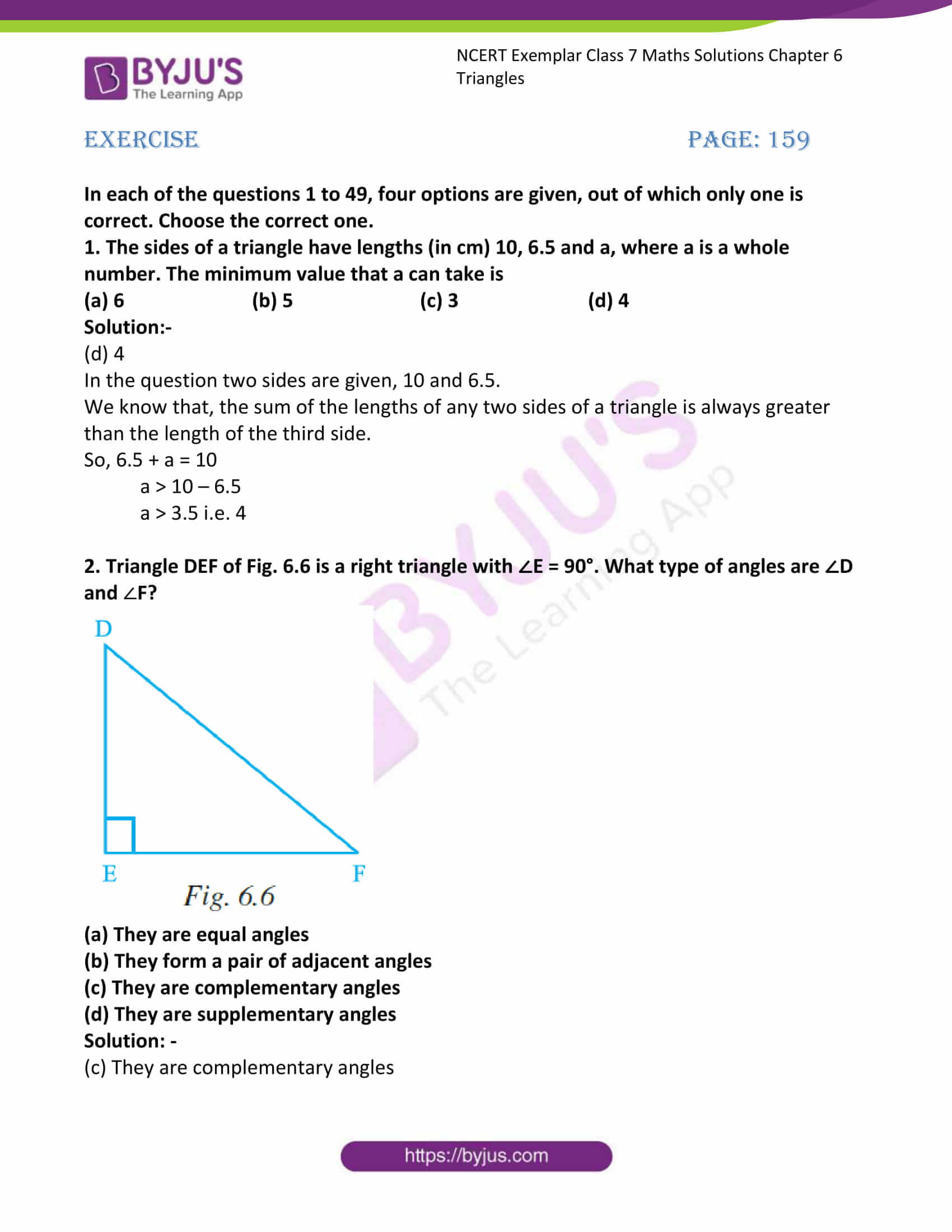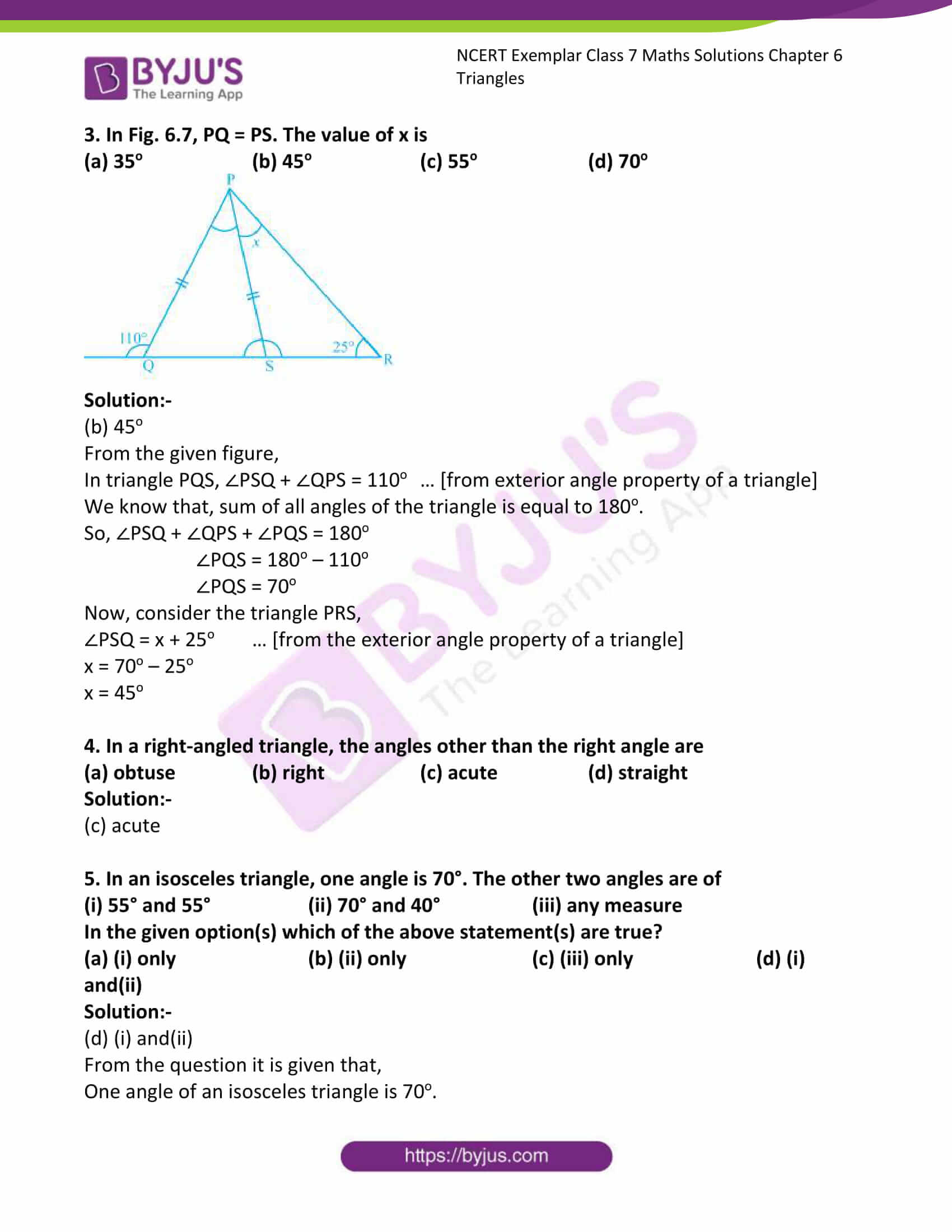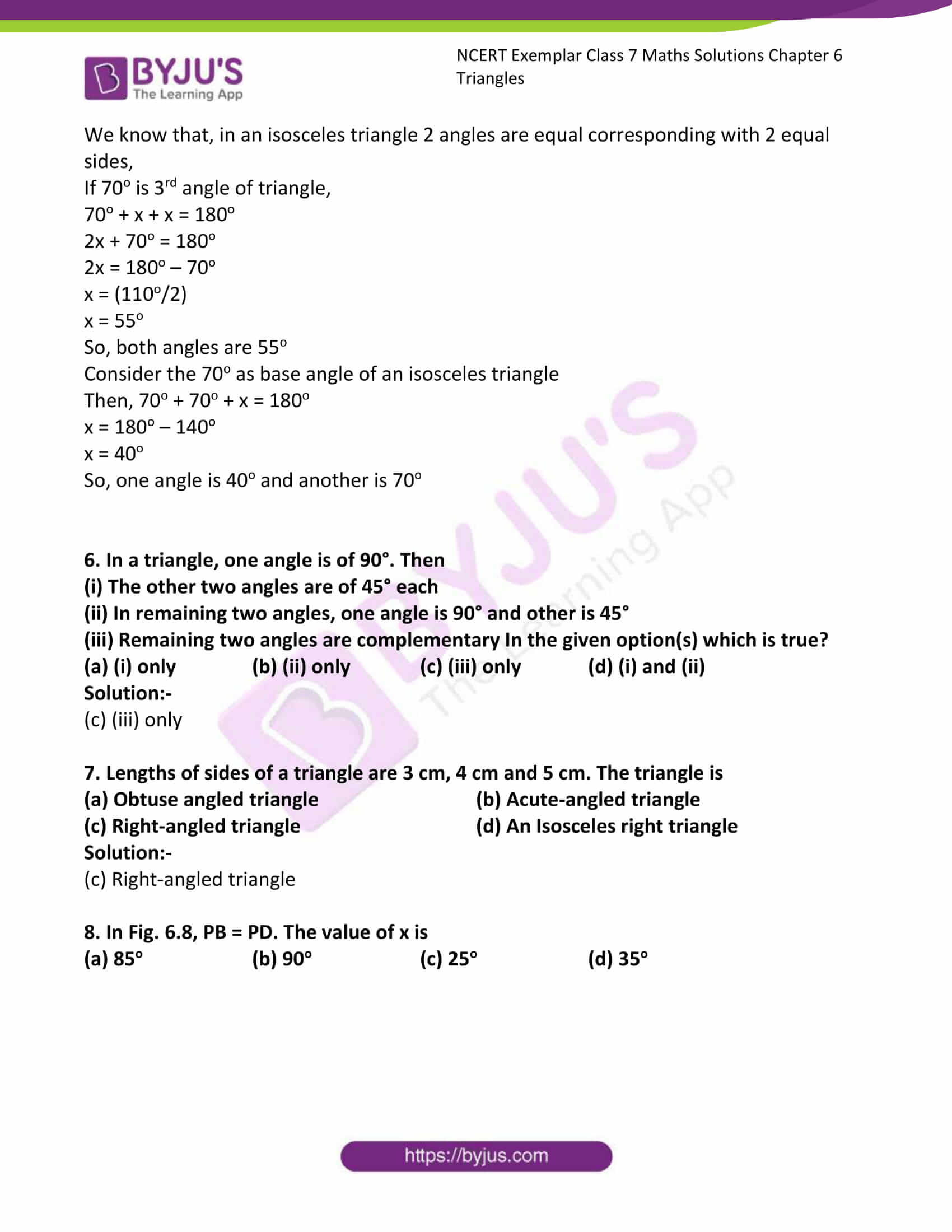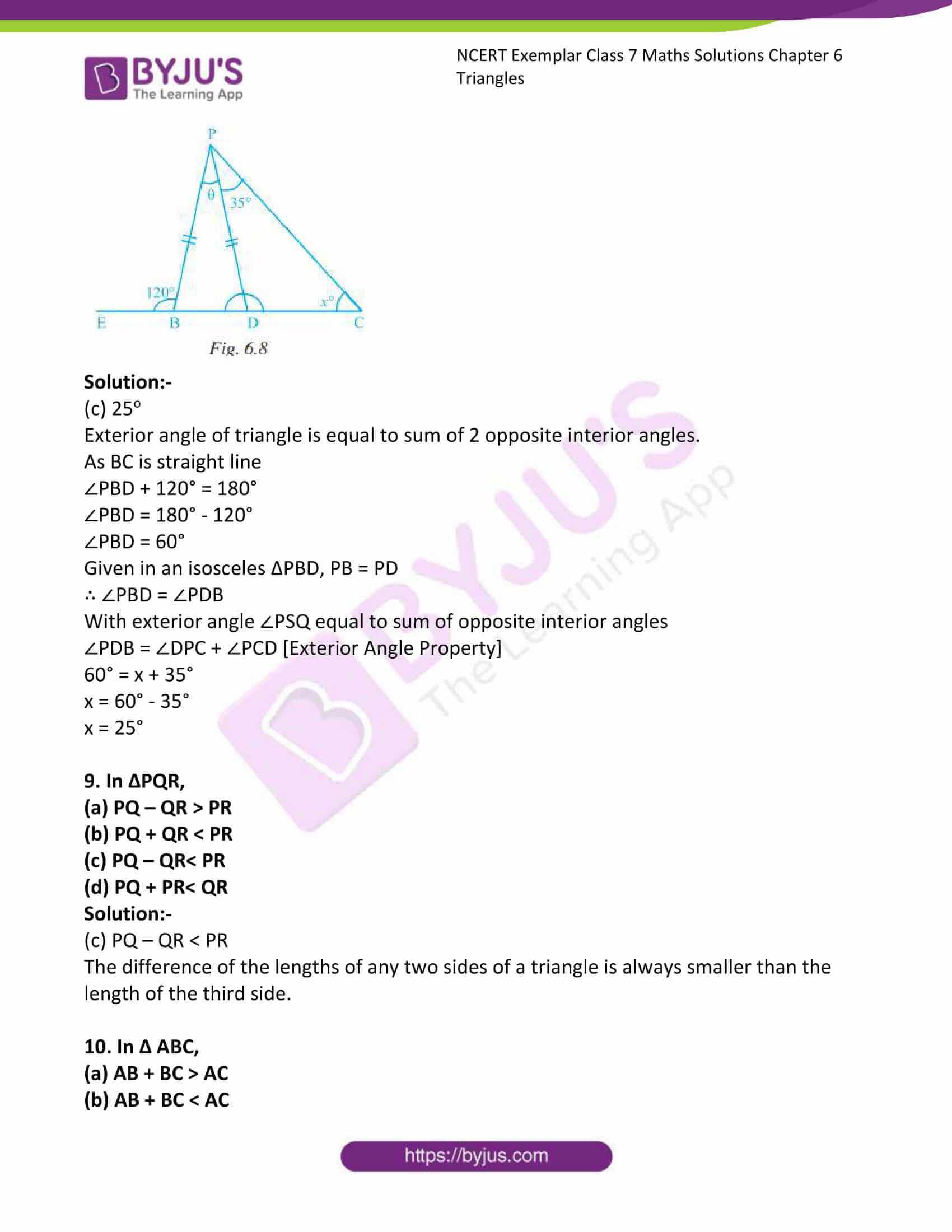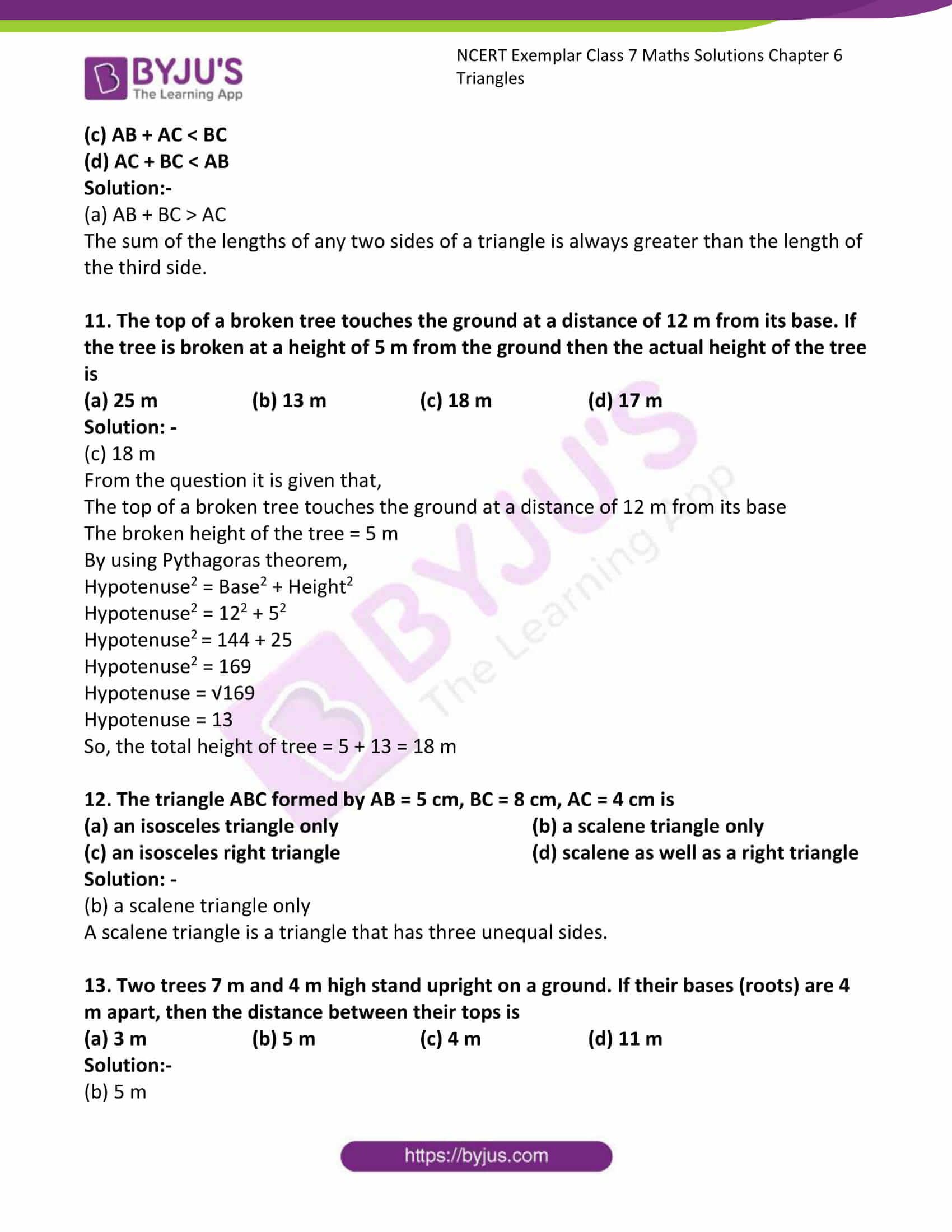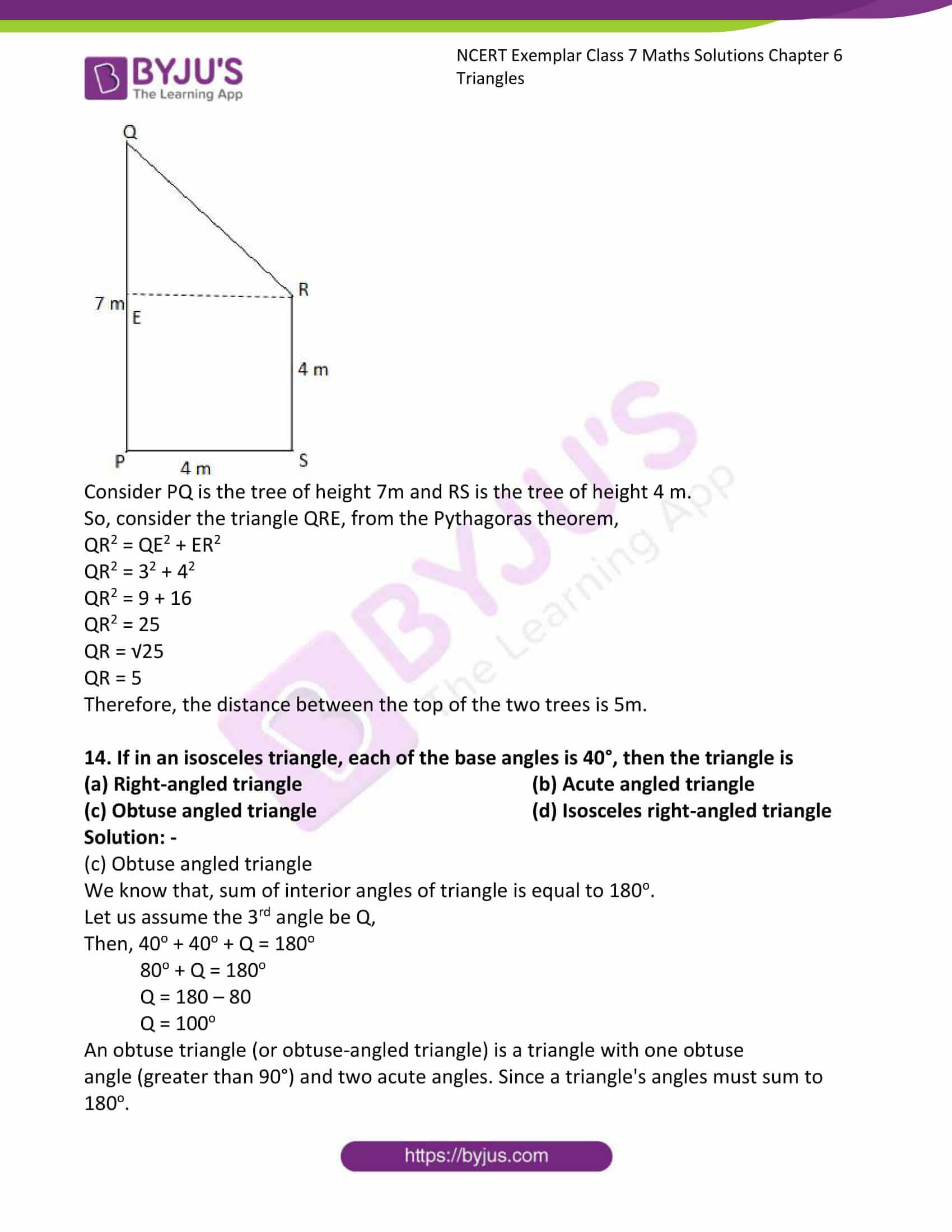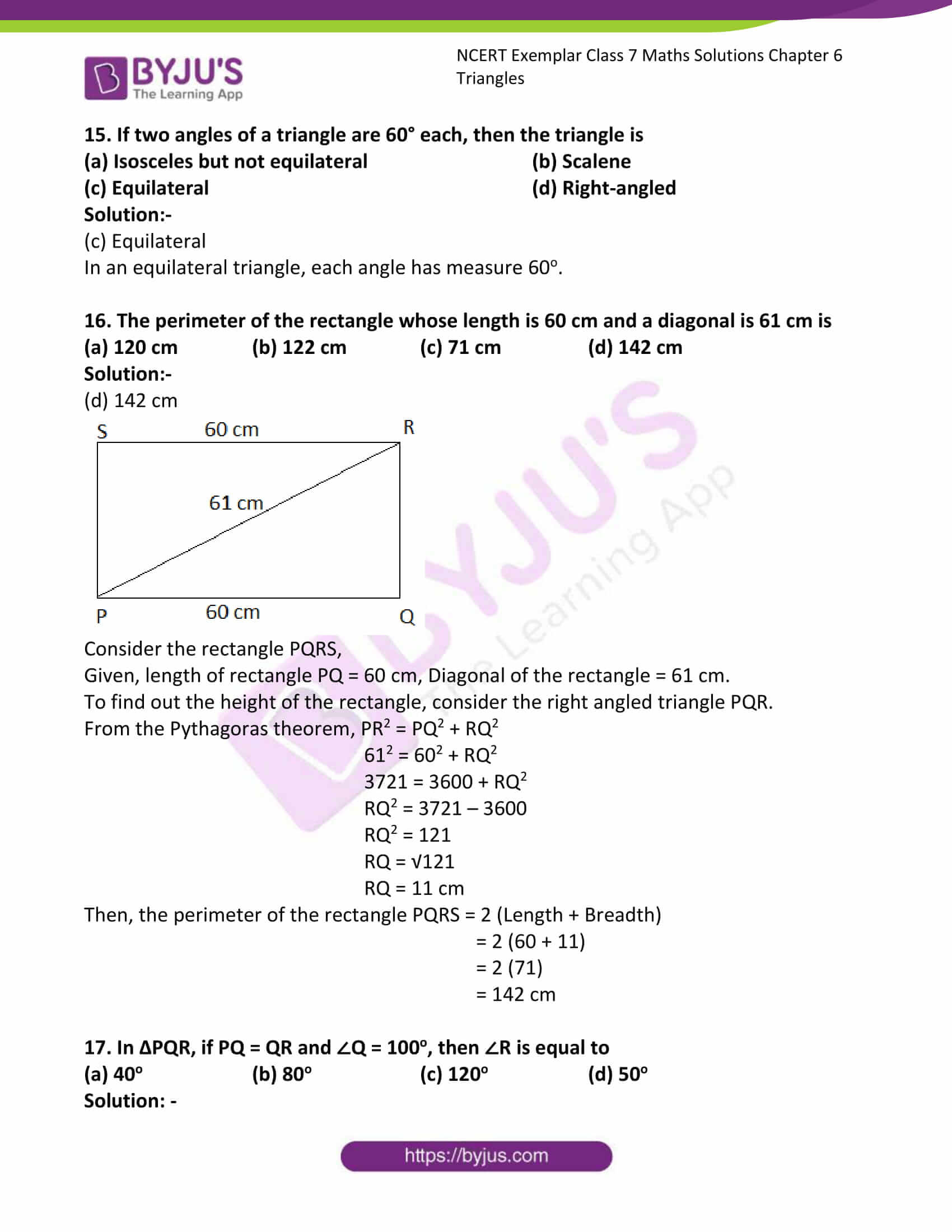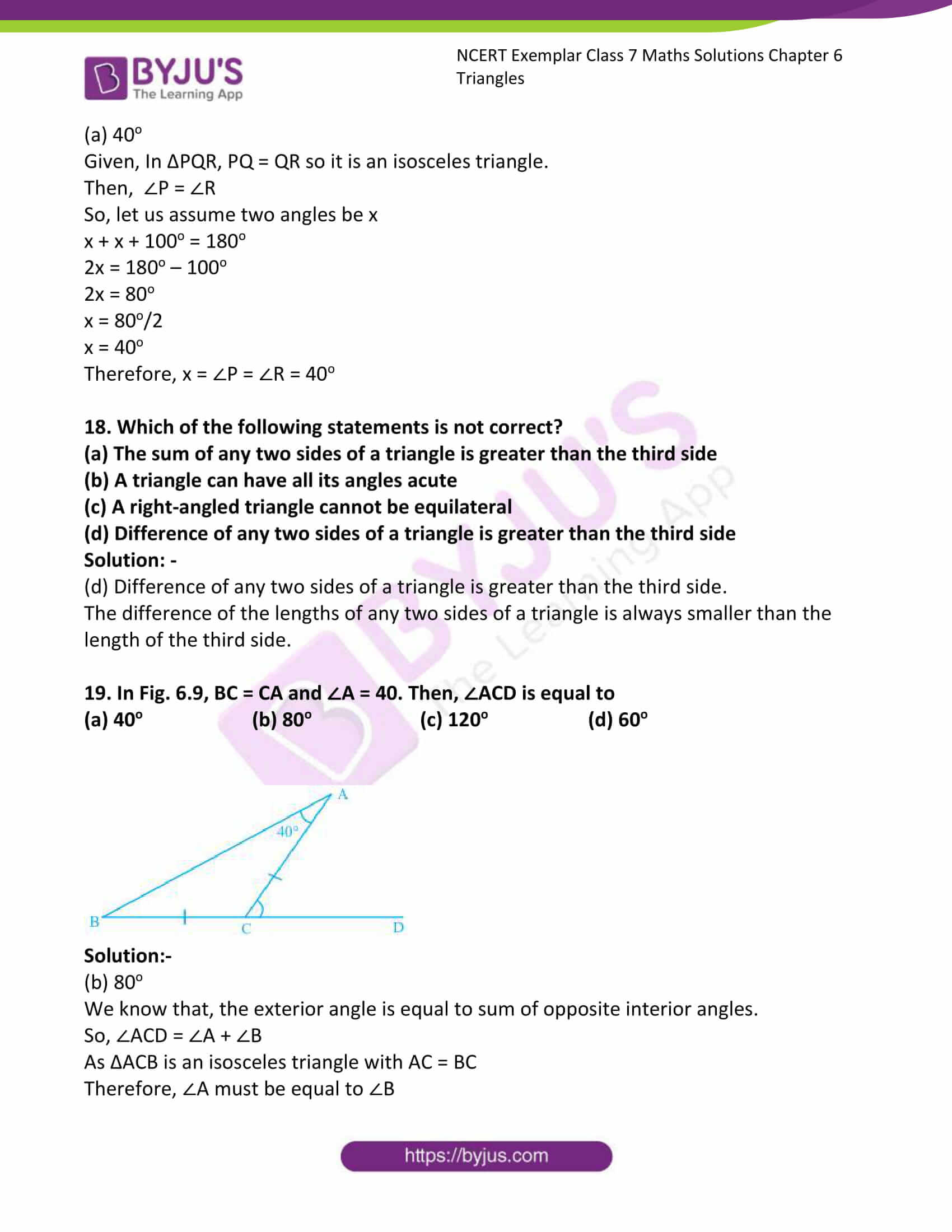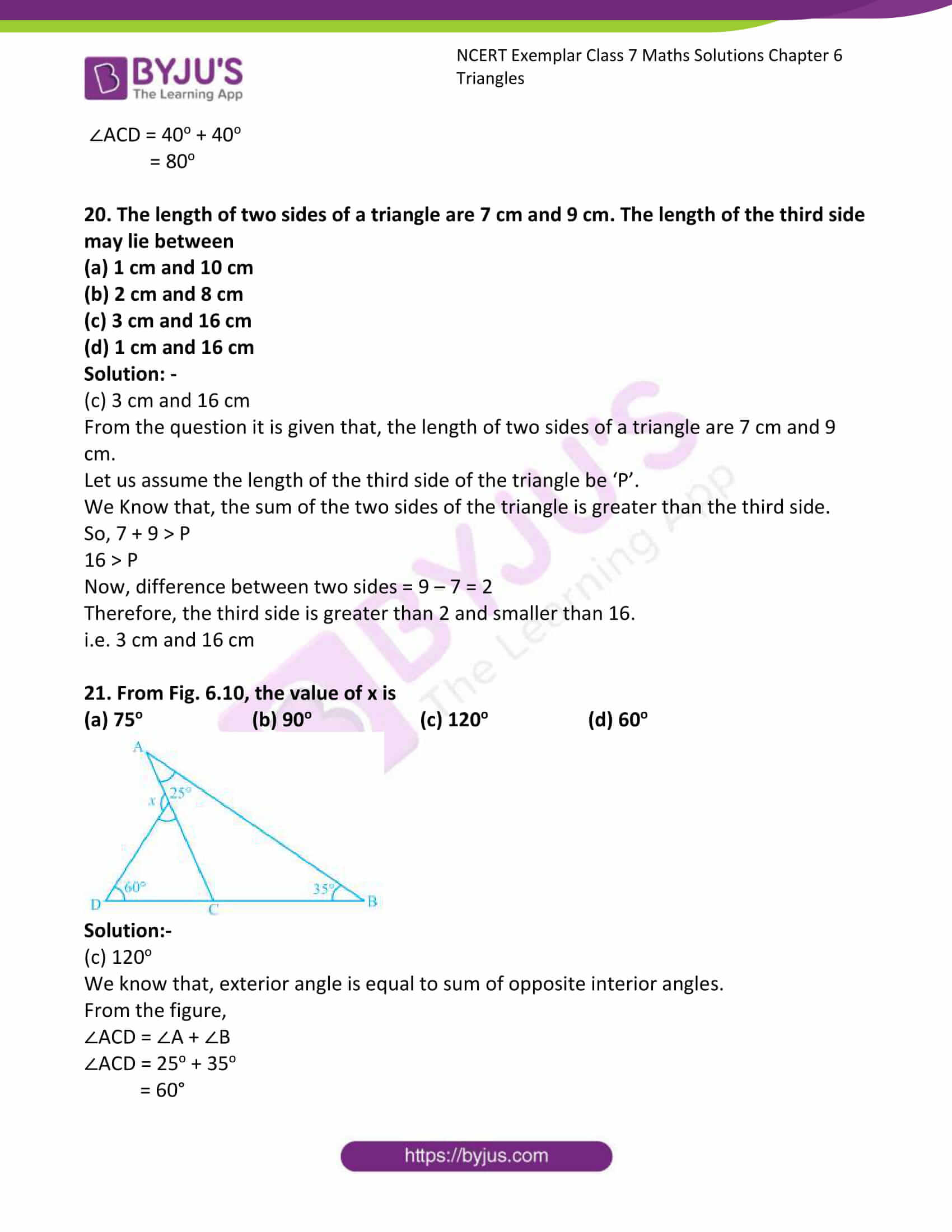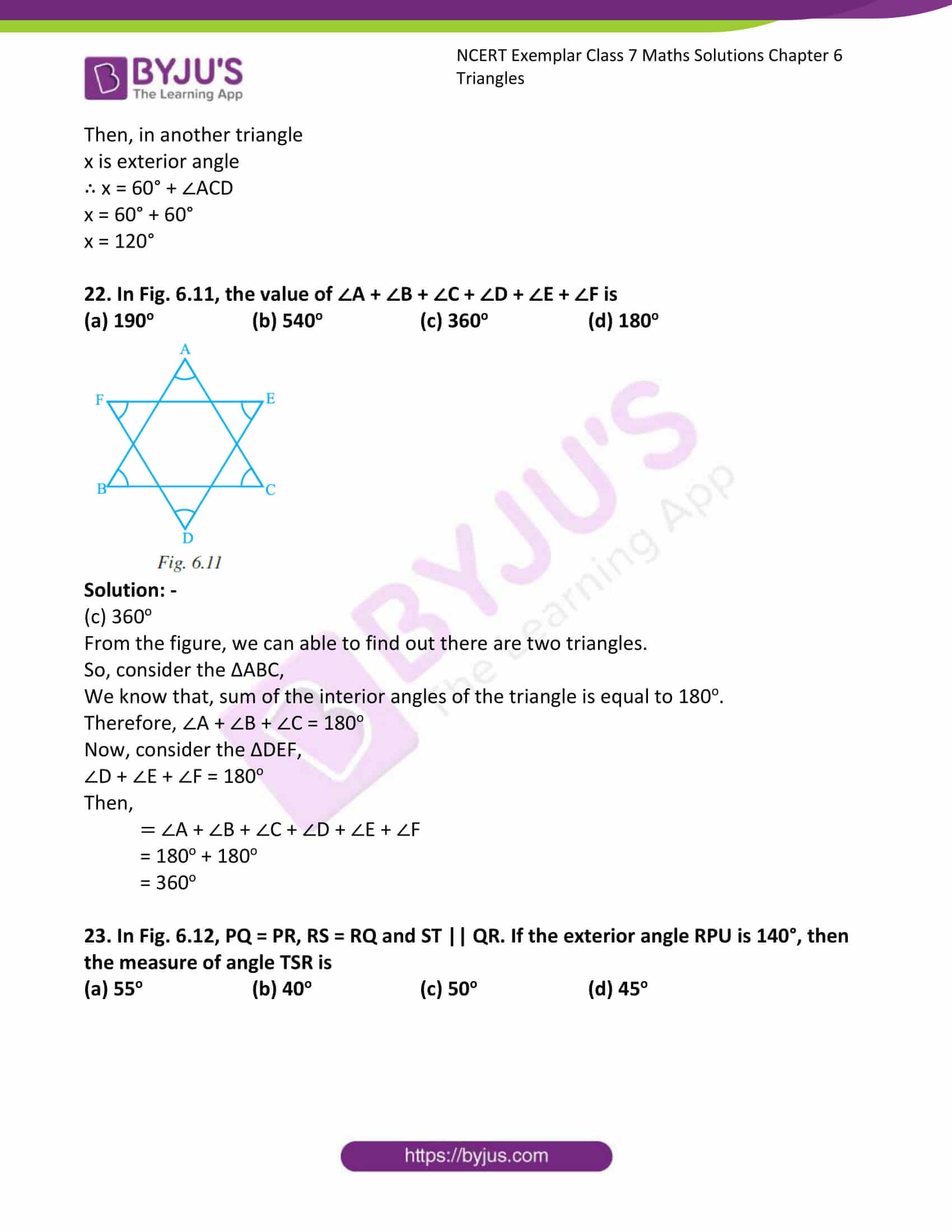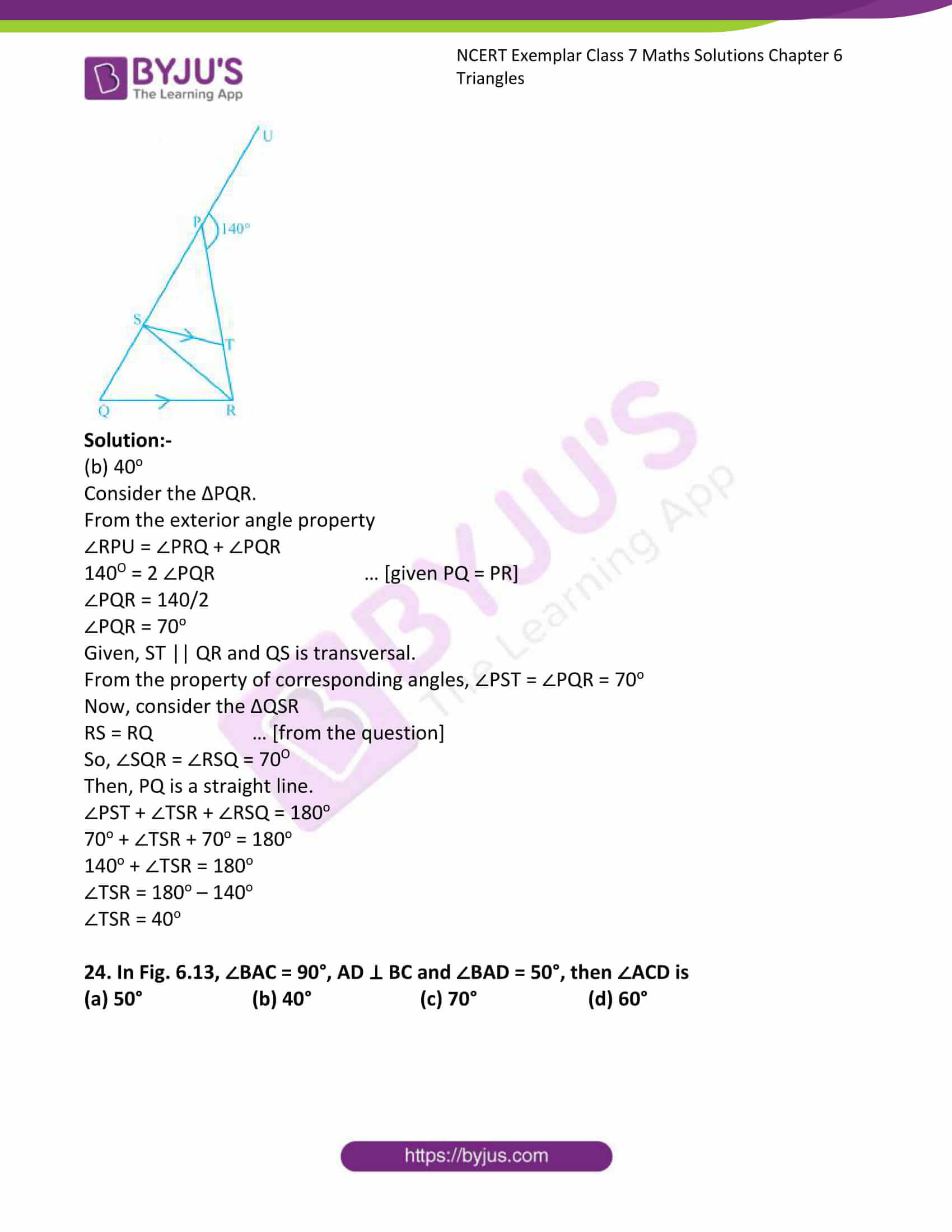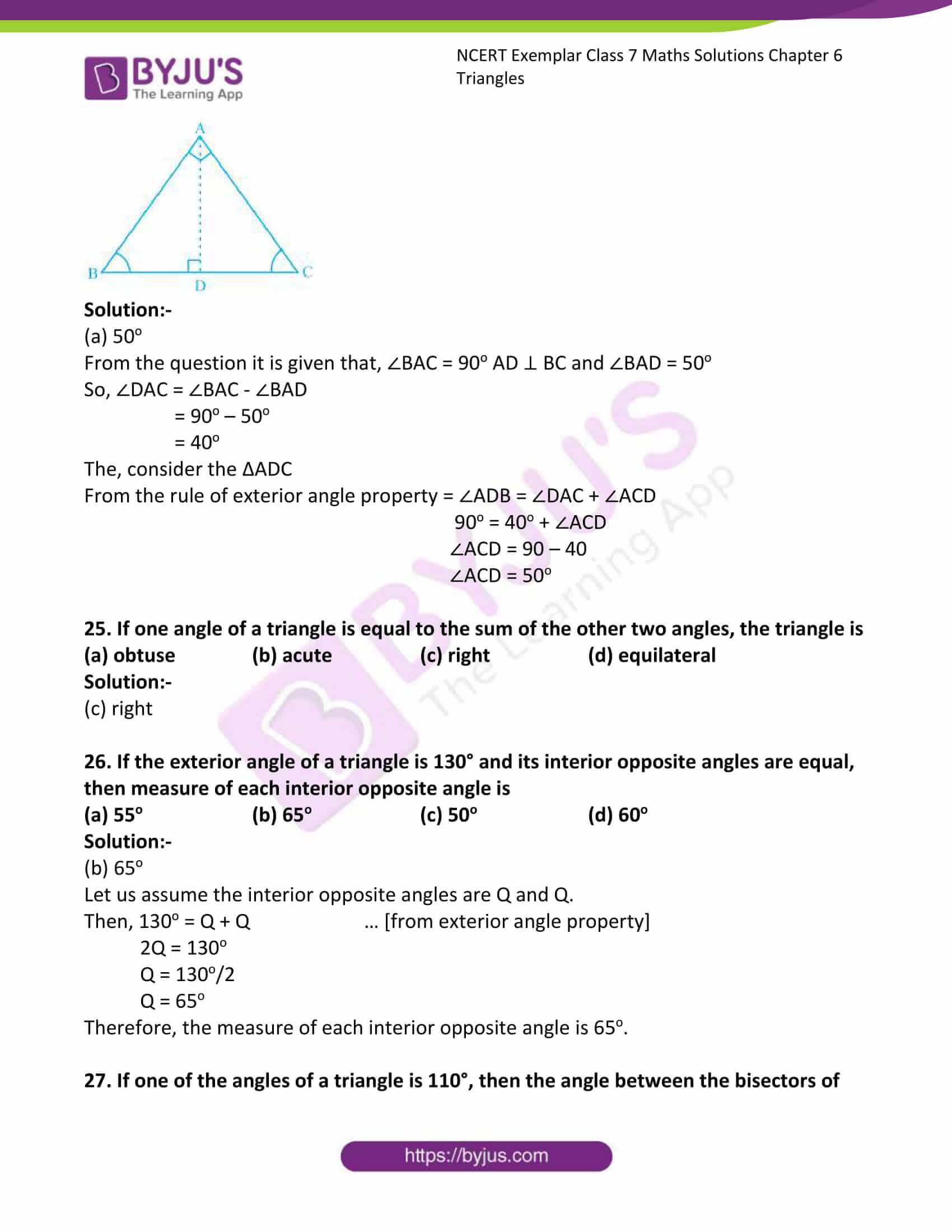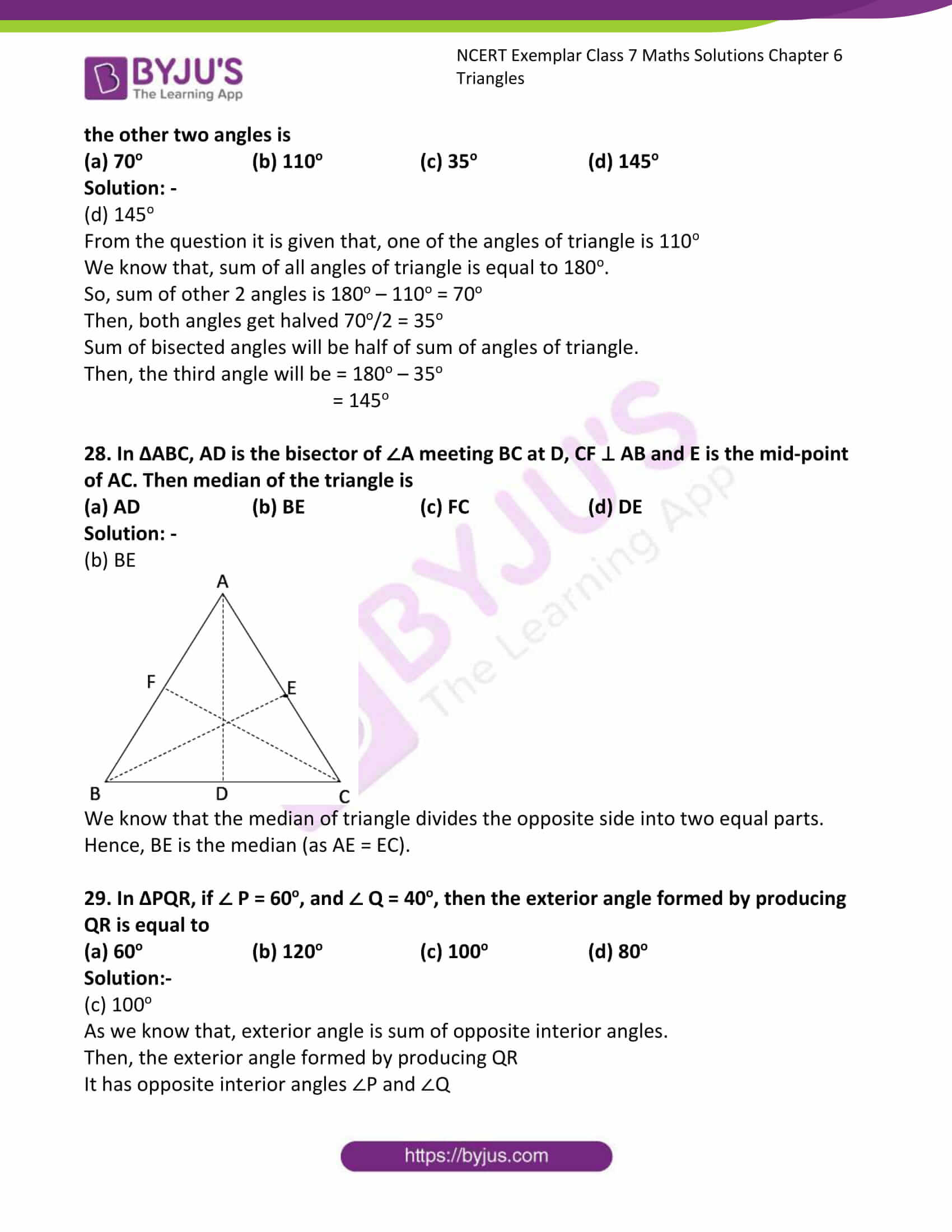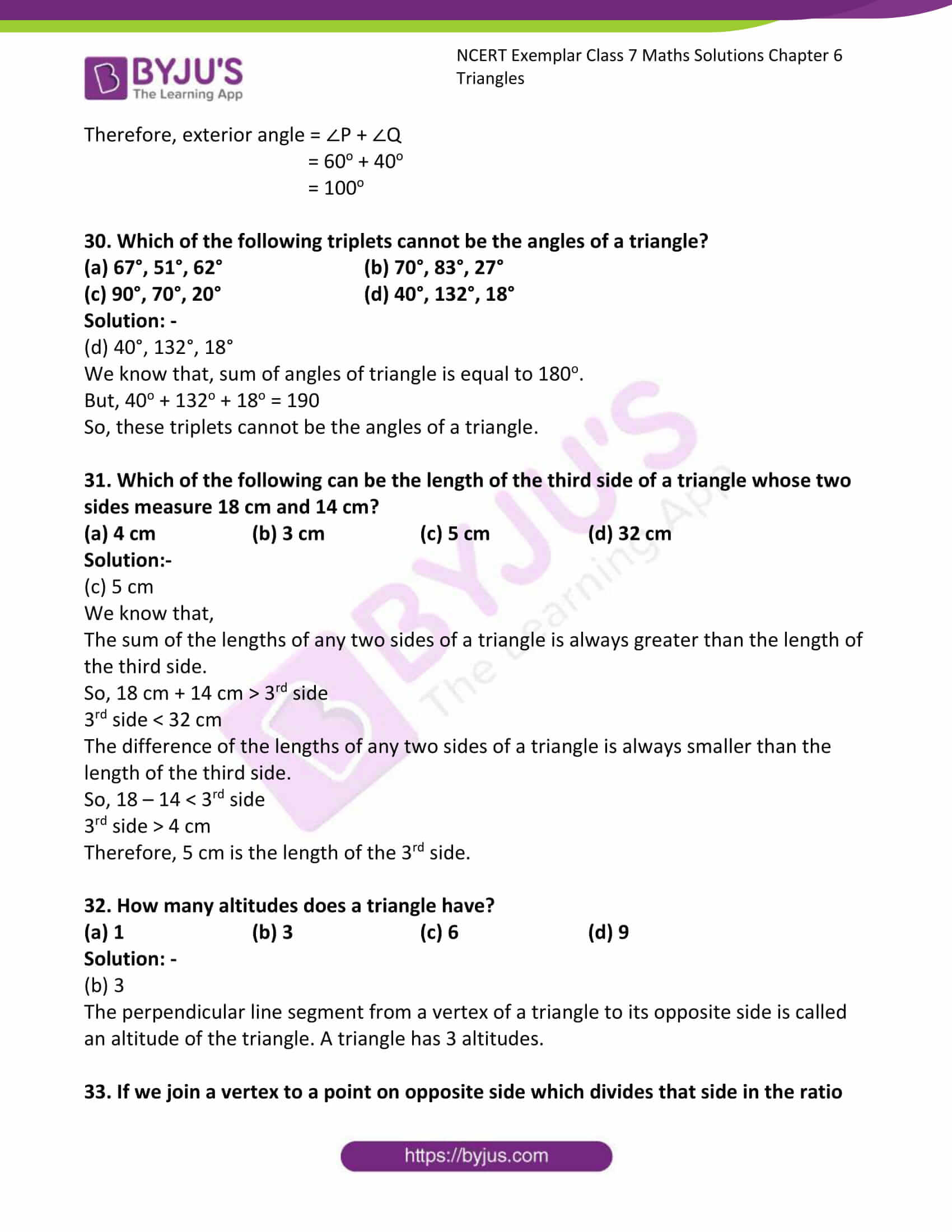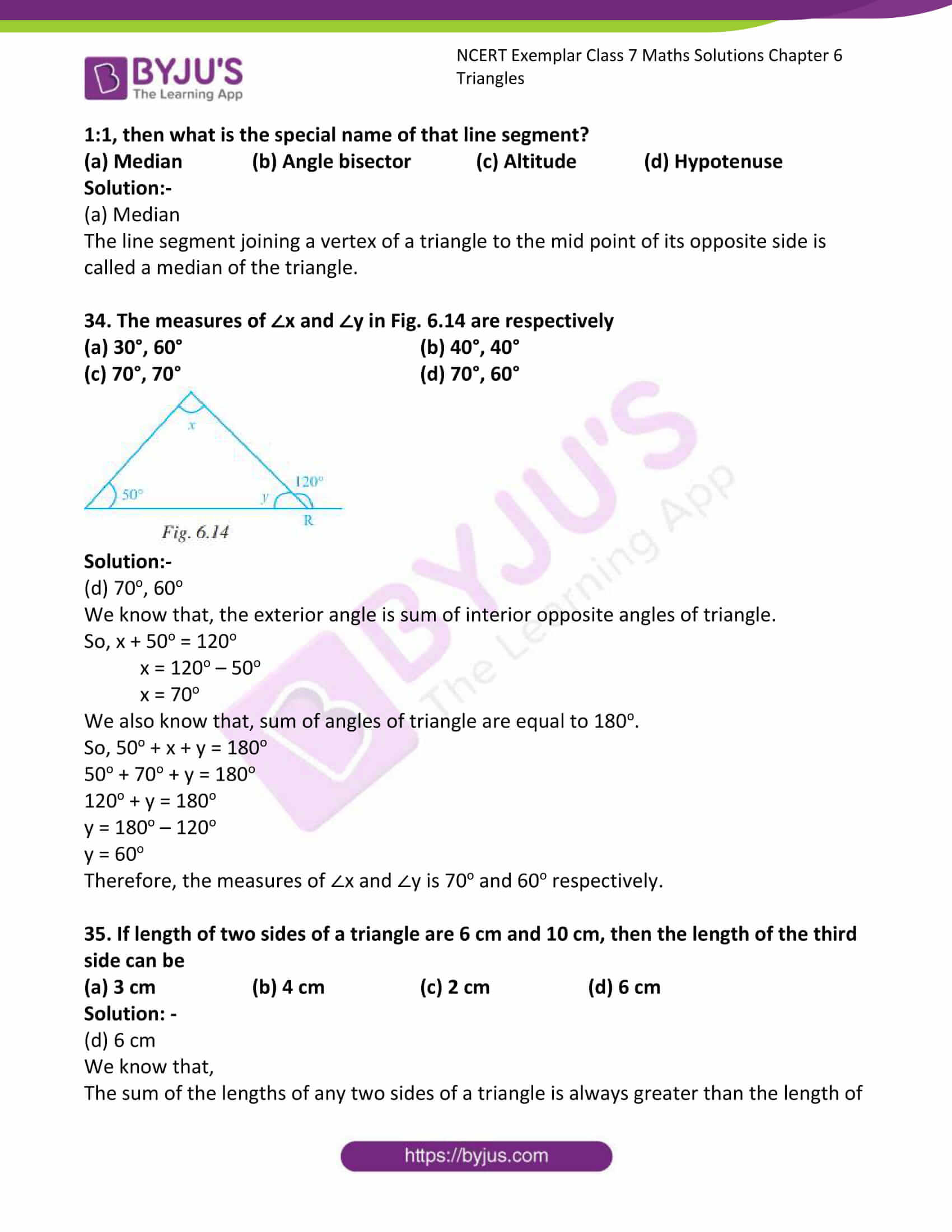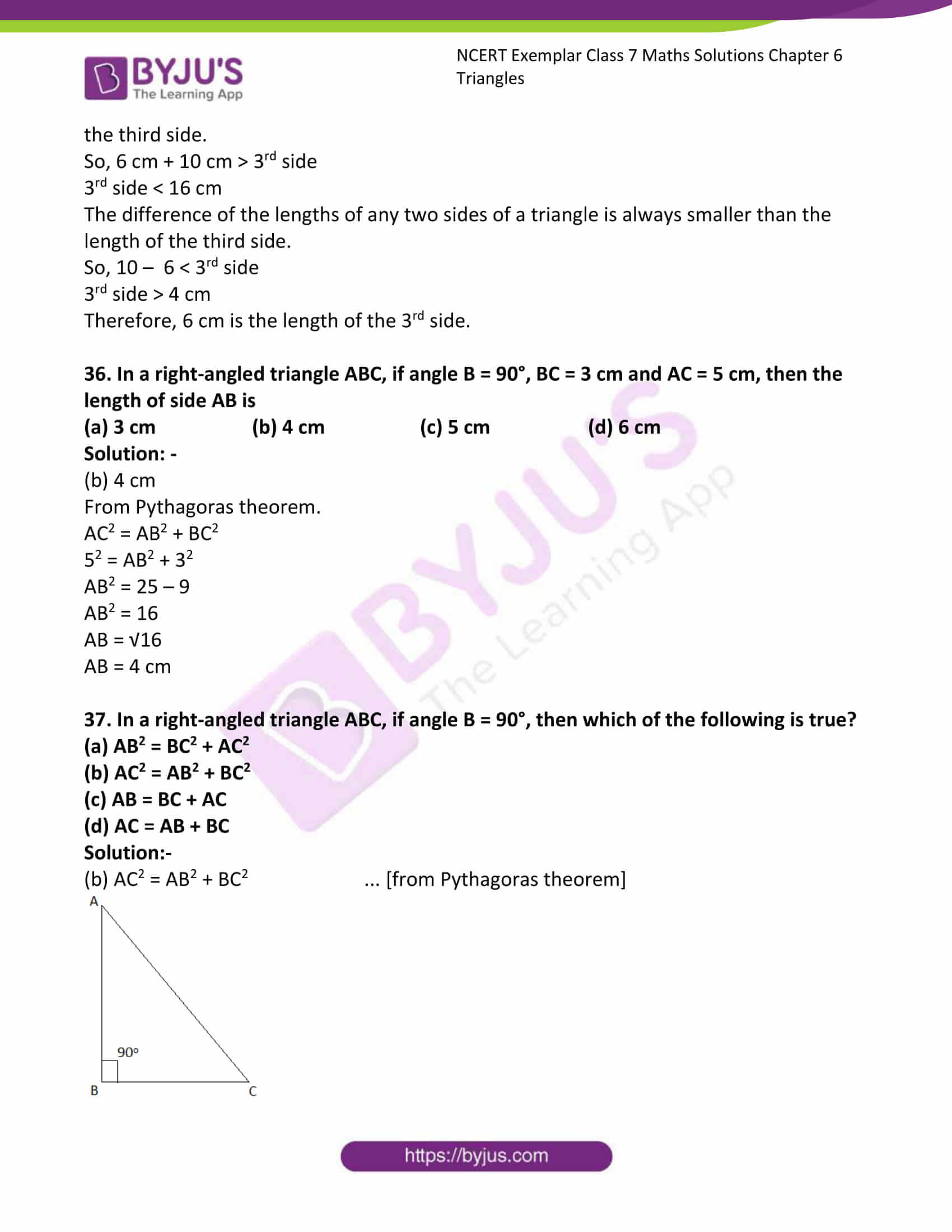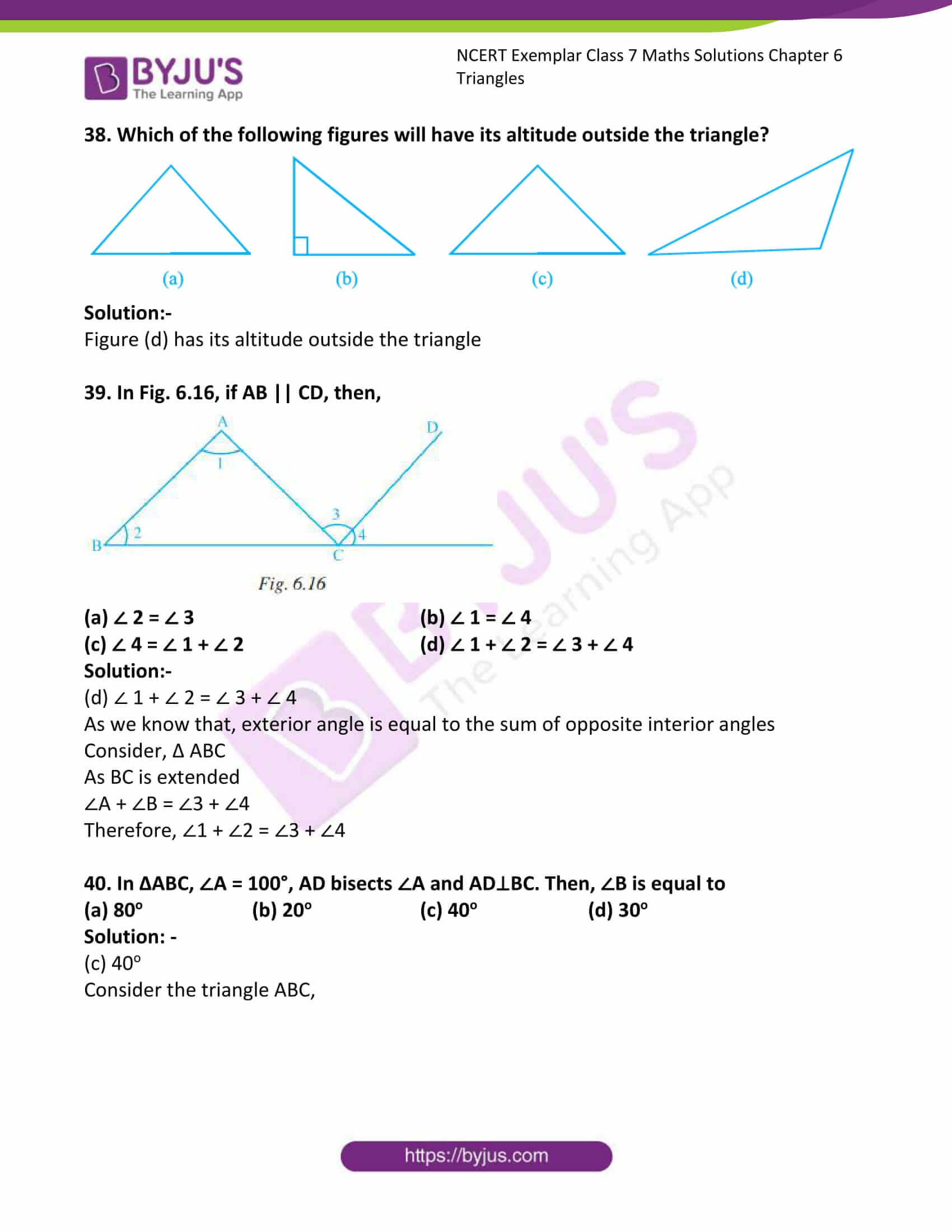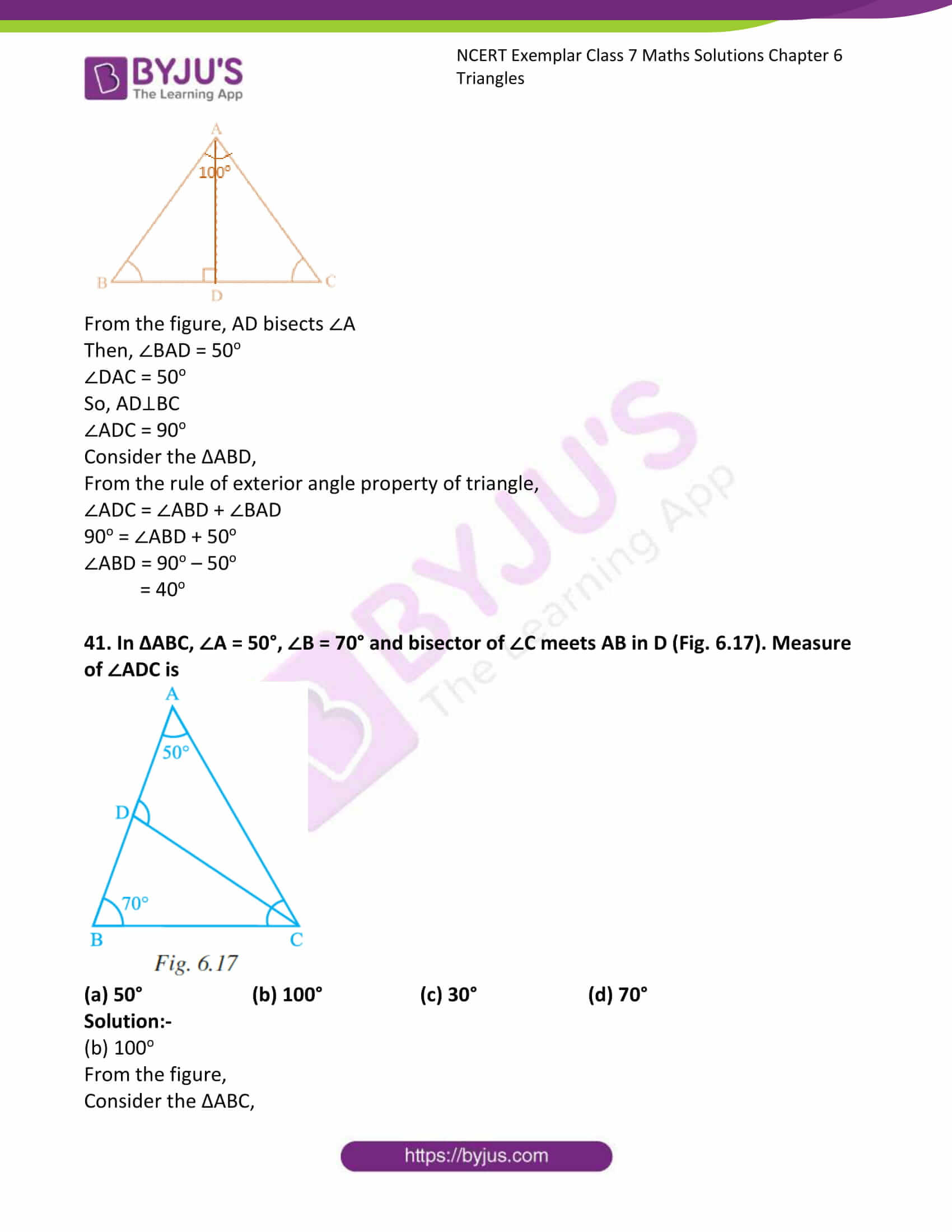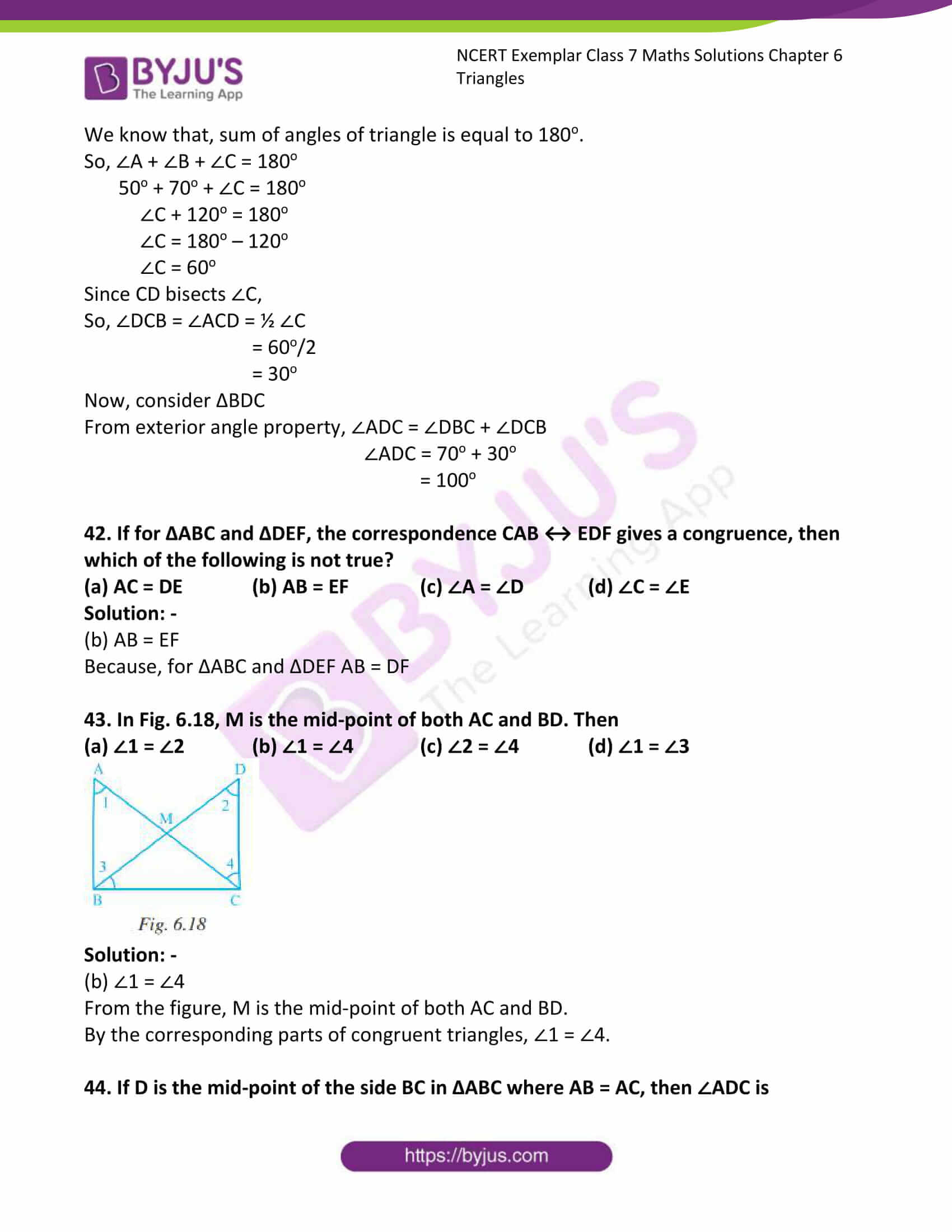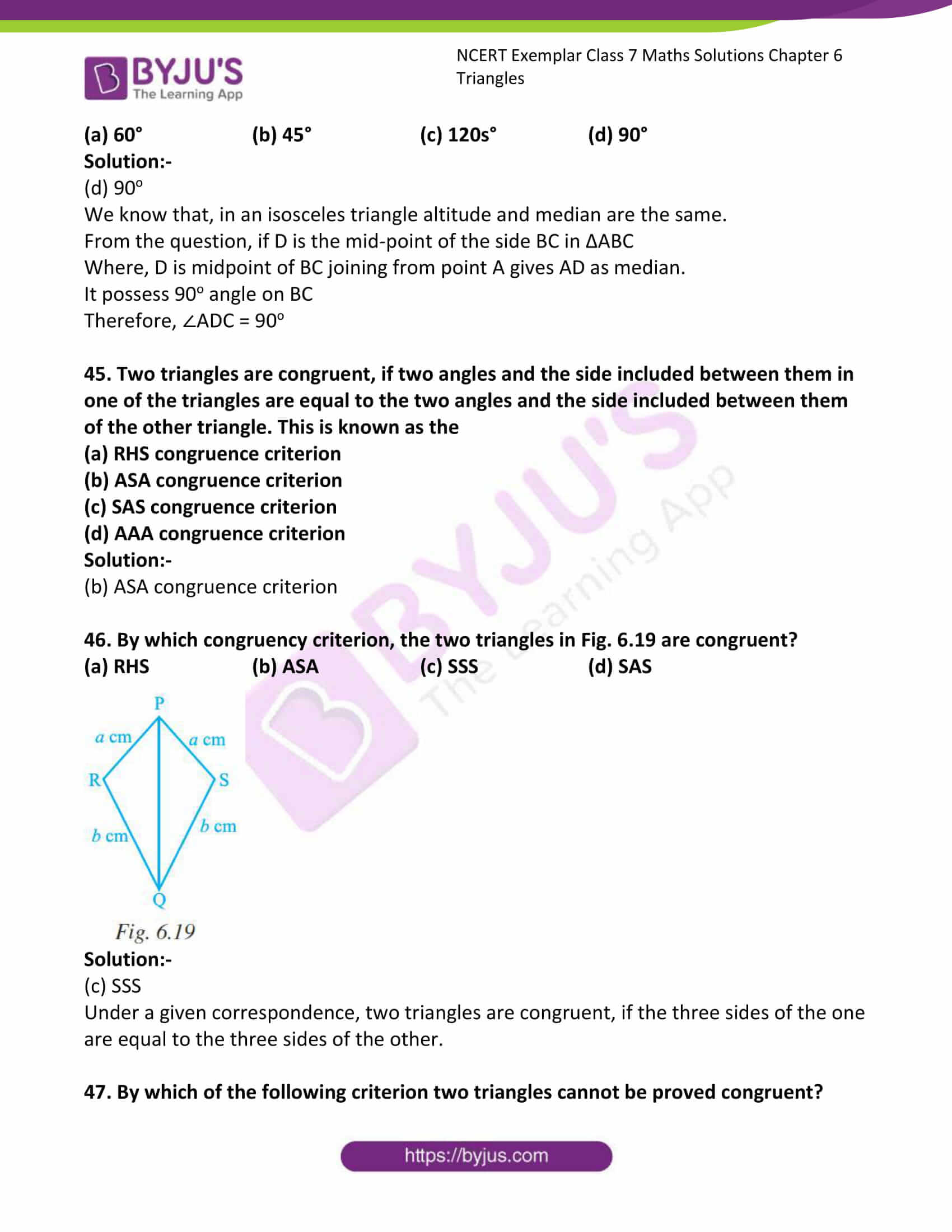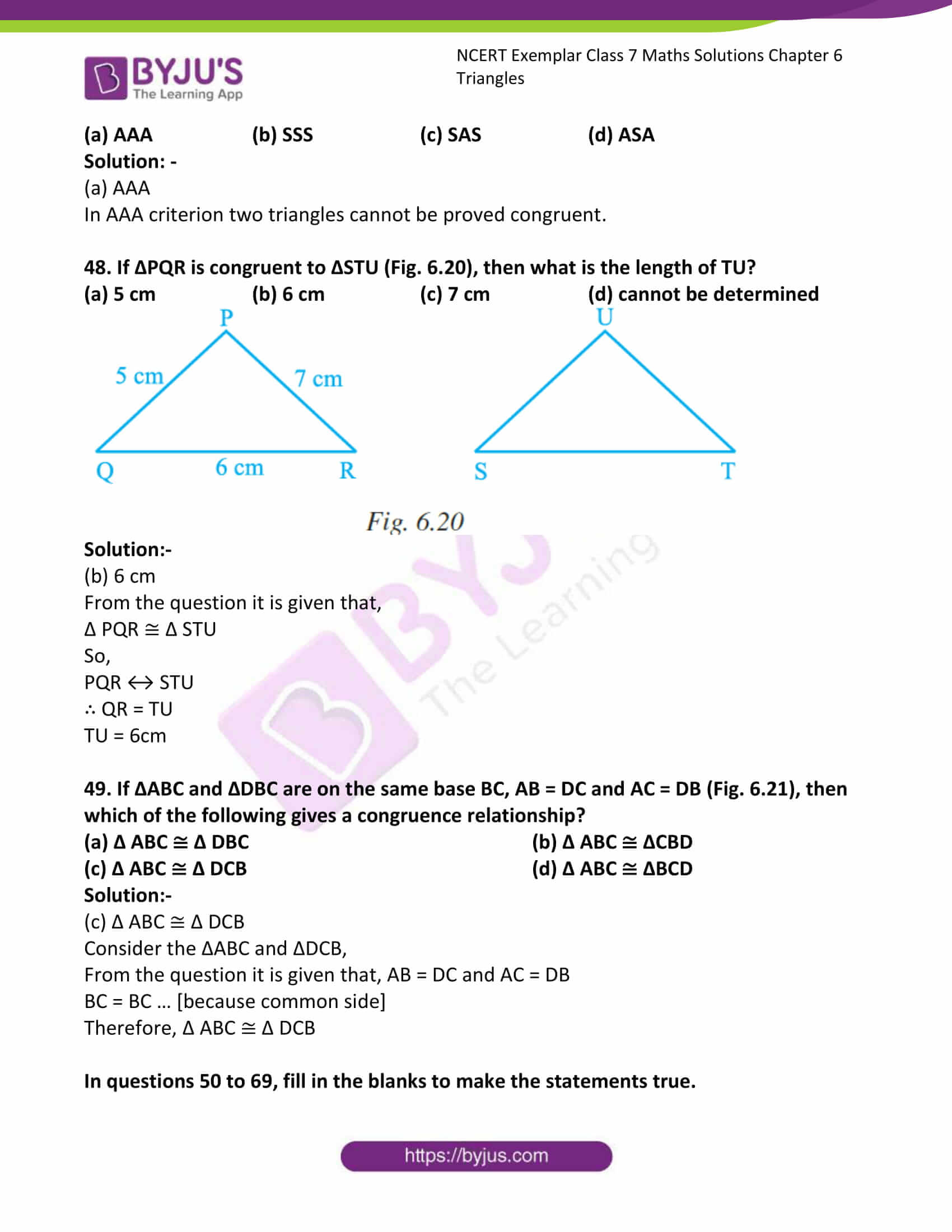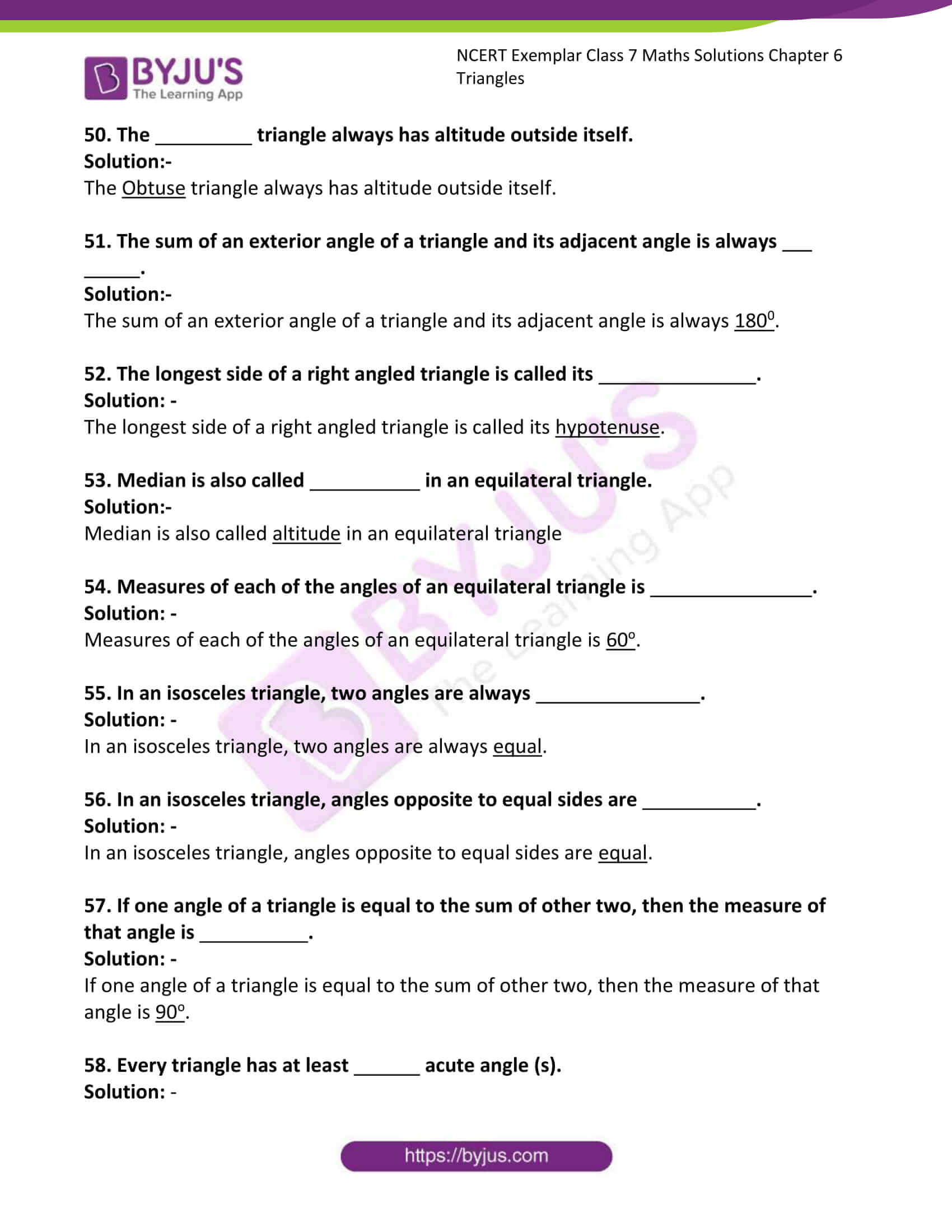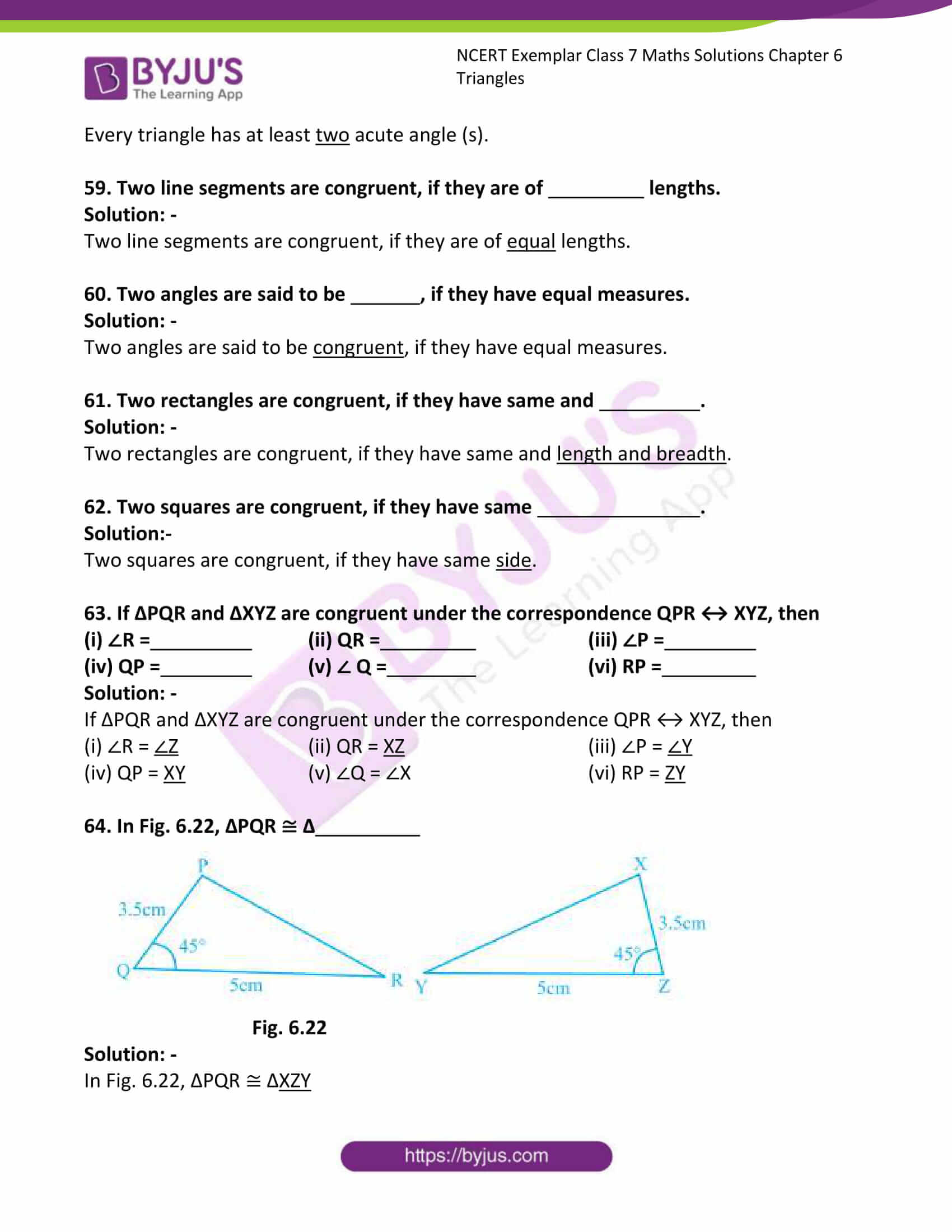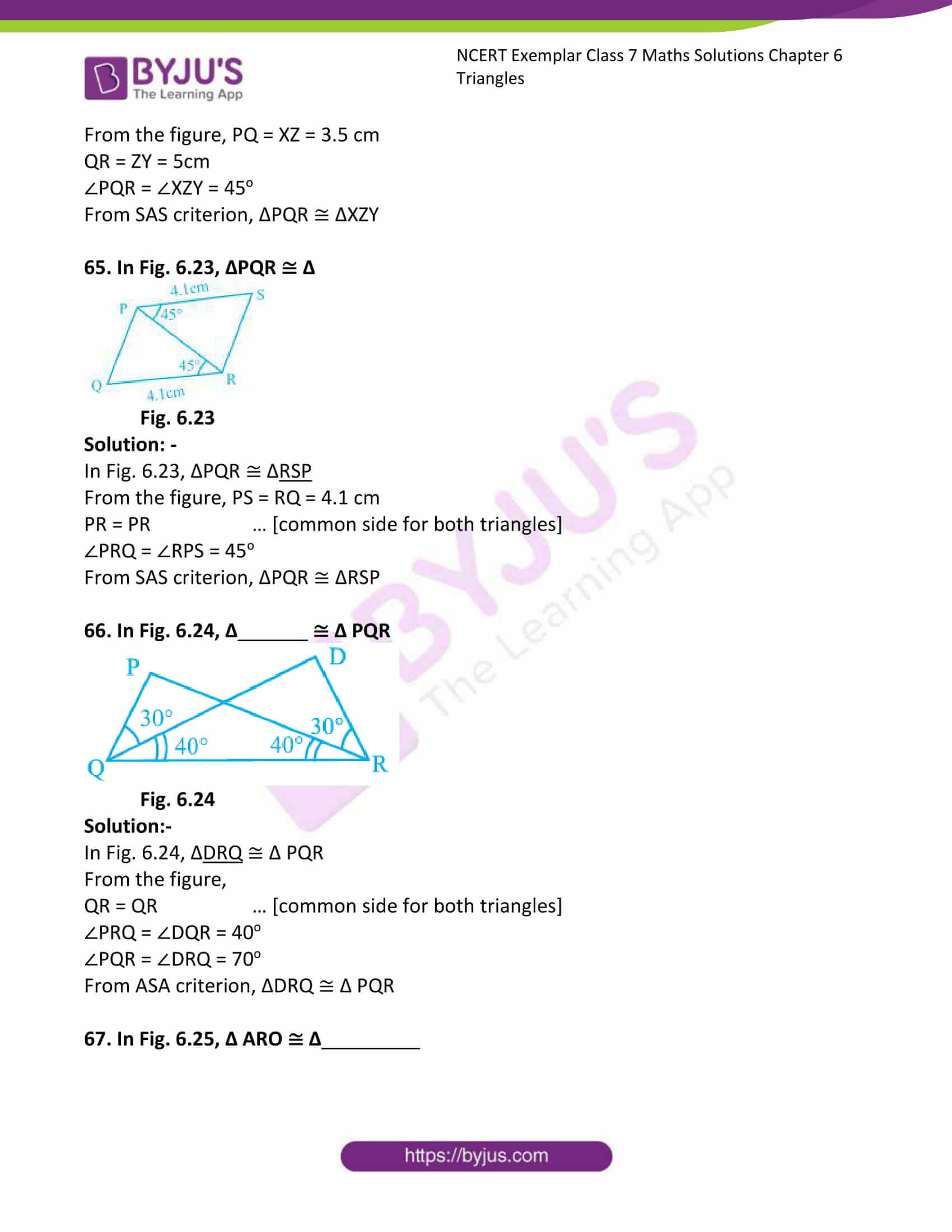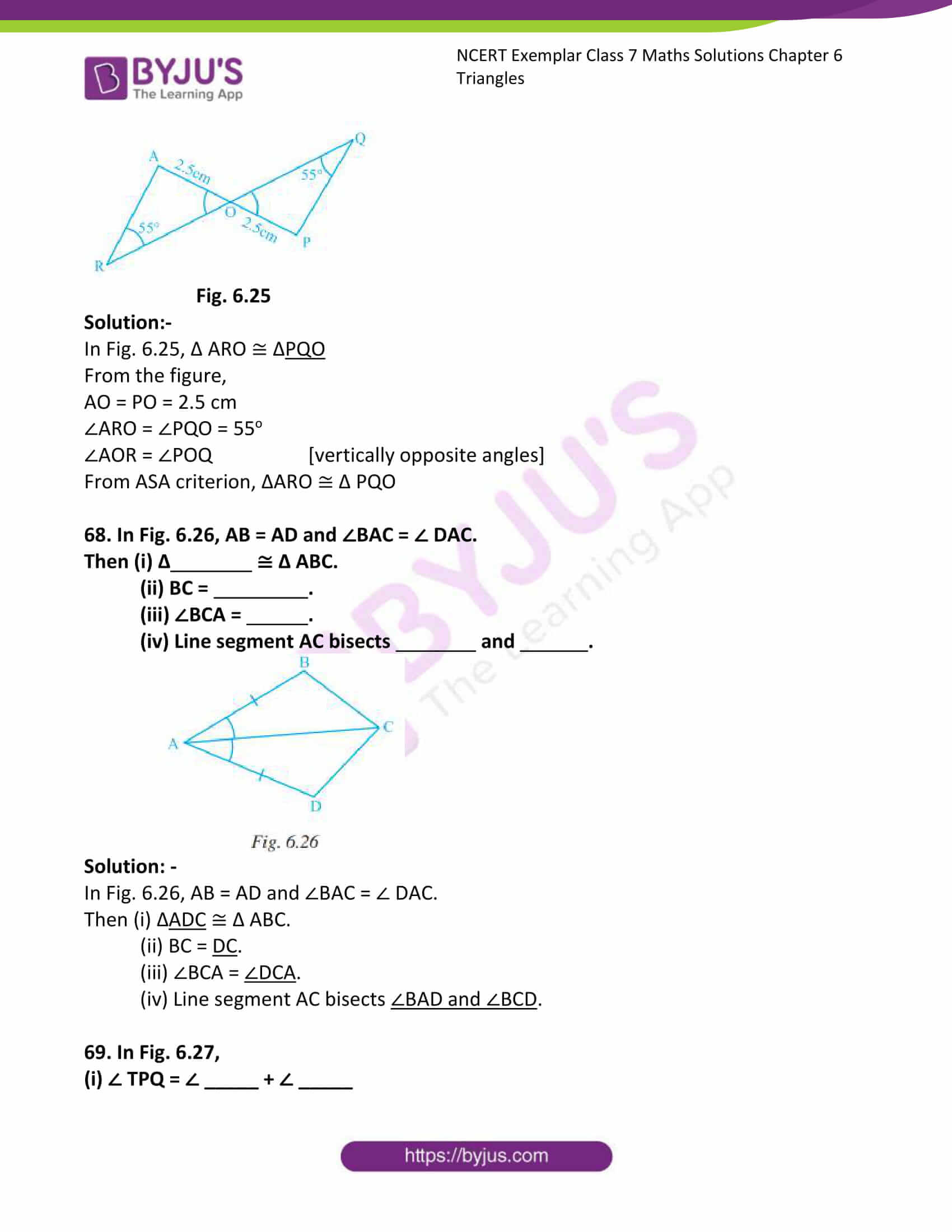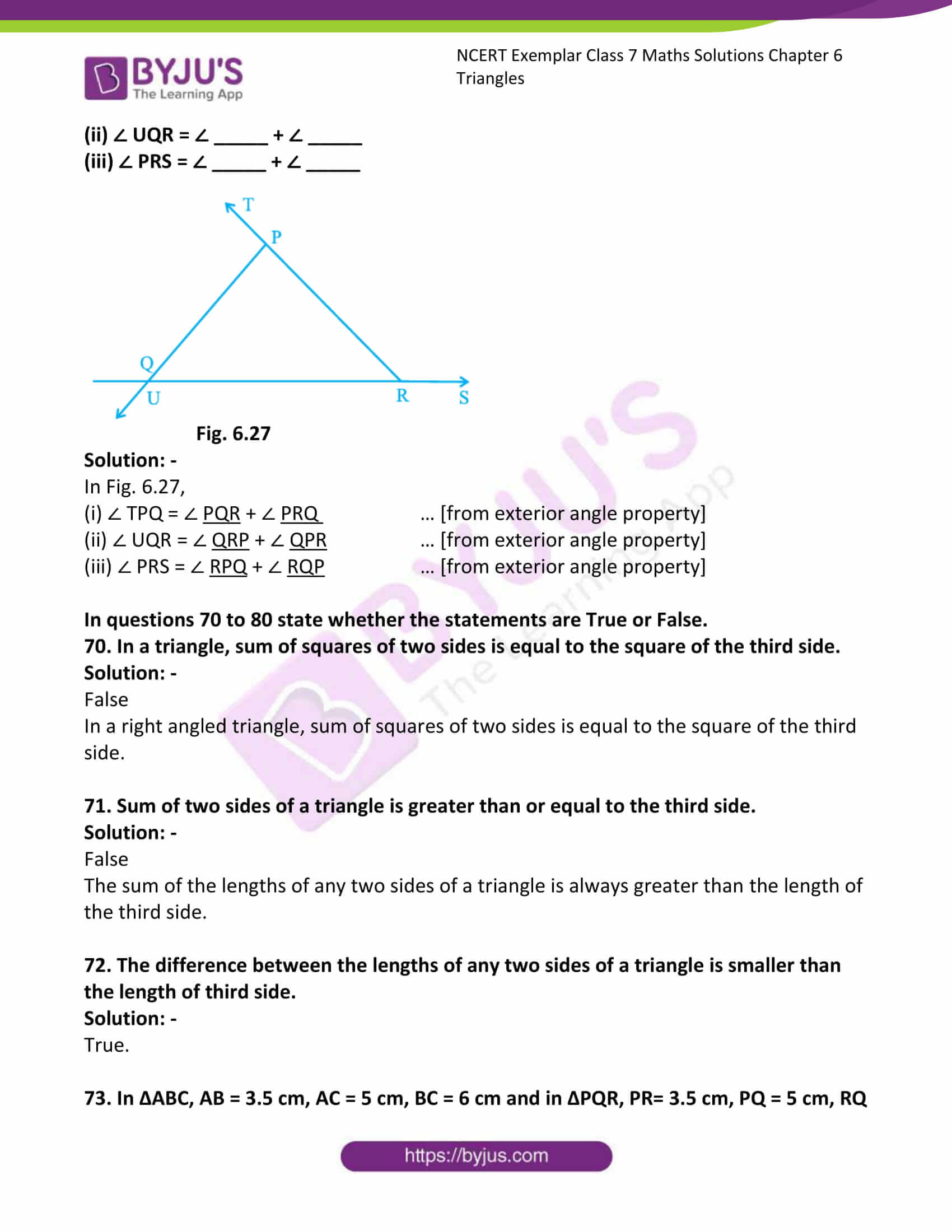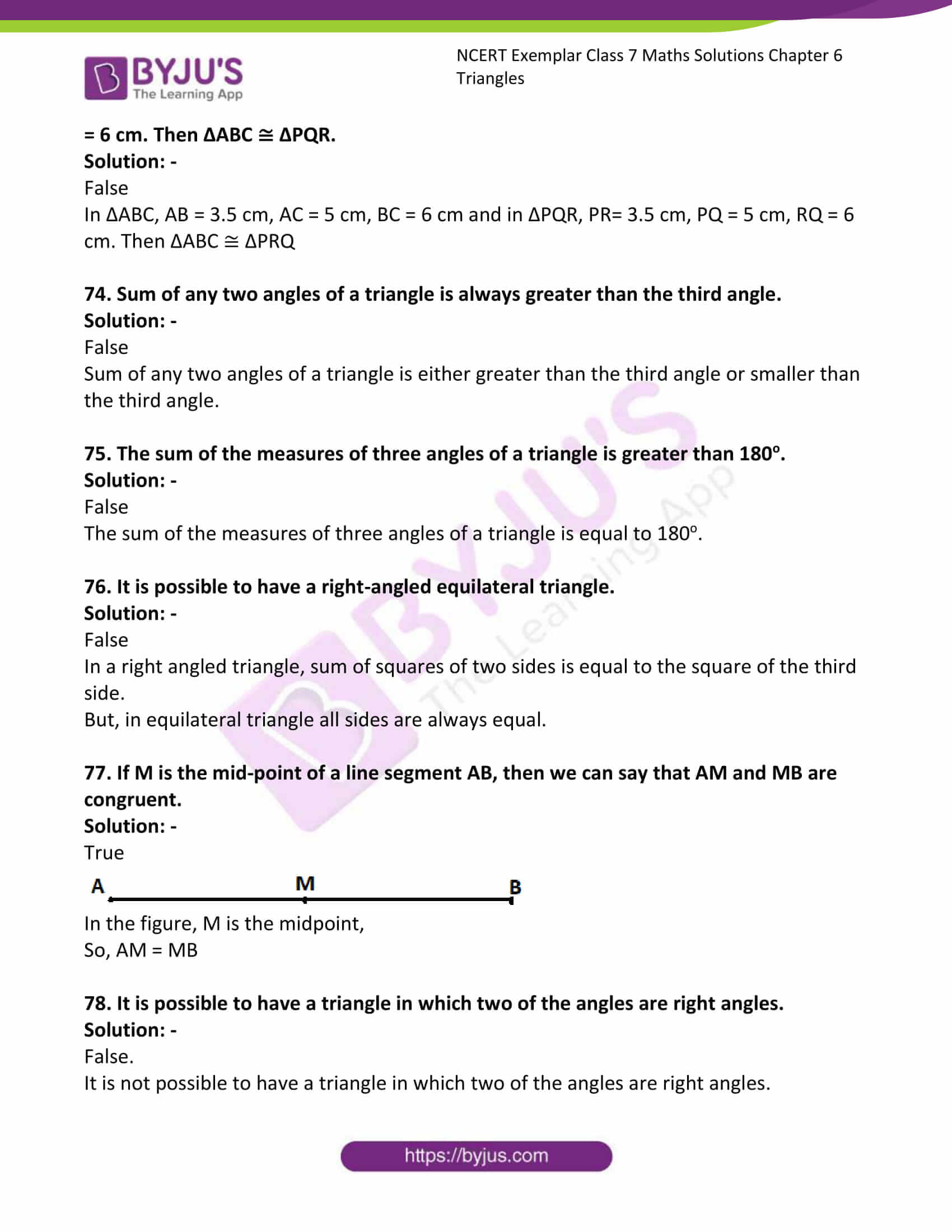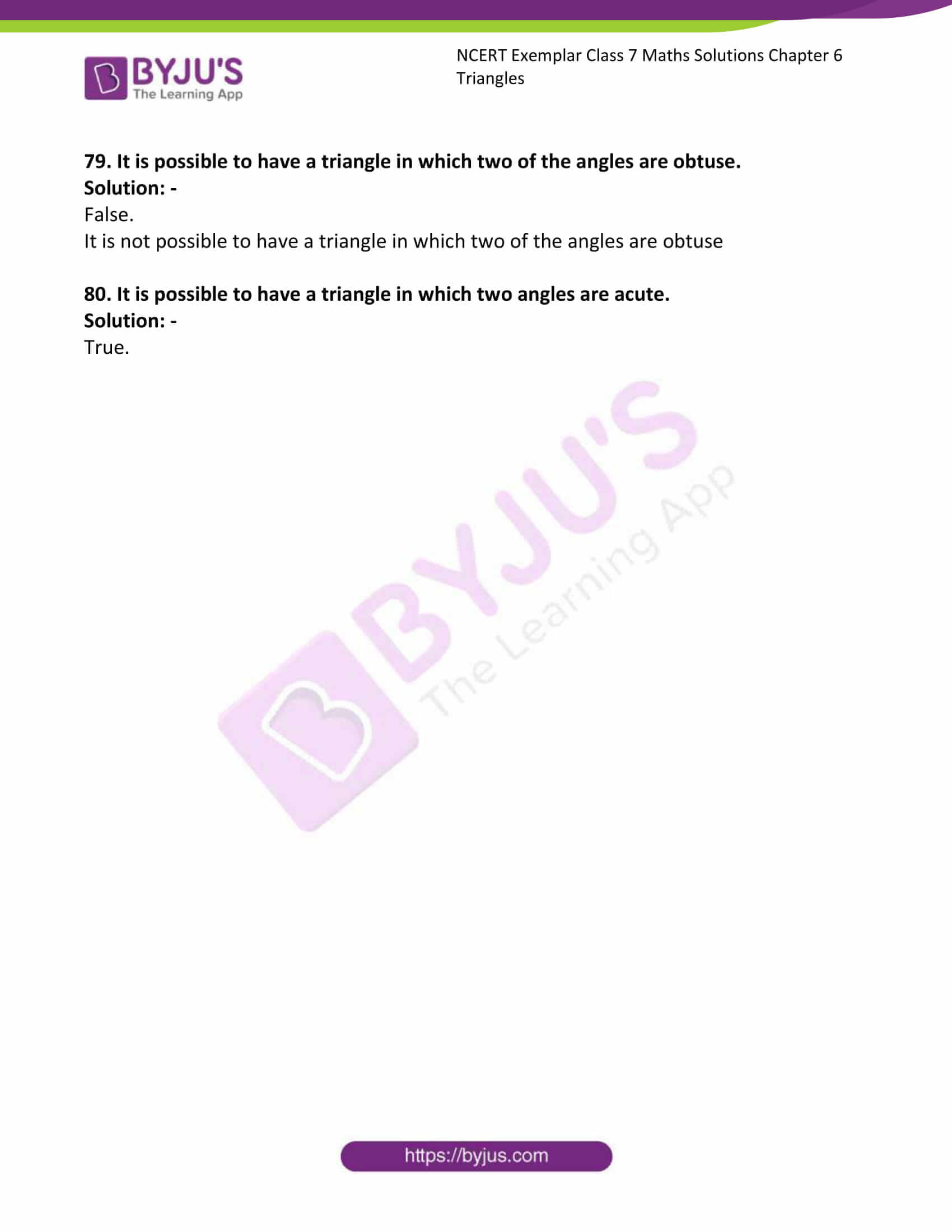### Access Answers to Maths NCERT Exemplar Solutions for Class 7 Chapter 6 Triangles

Exercise Page: 159

In each of the questions 1 to 49, four options are given, out of which only one is correct. Choose the correct one.

1. The sides of a triangle have lengths (in cm) 10, 6.5 and a, where a is a whole number. The minimum value that a can take is

(a) 6 (b) 5 (c) 3 (d) 4

Solution:-

(d) 4

In the question two sides are given, 10 and 6.5.

We know that, the sum of the lengths of any two sides of a triangle is always greater than the length of the third side.

So, 6.5 + a = 10

a > 10 – 6.5

a > 3.5 i.e. 4

2. Triangle DEF of Fig. 6.6 is a right triangle with ∠E = 90°. What type of angles are ∠D and F?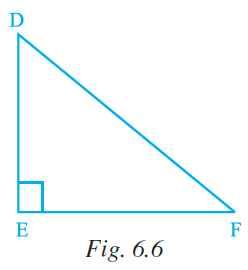(a) They are equal angles

(b) They form a pair of adjacent angles

(c) They are complementary angles

(d) They are supplementary angles

Solution: –

(c) They are complementary angles

3. In Fig. 6.7, PQ = PS. The value of x is

(a) 35o (b) 45o (c) 55o (d) 70o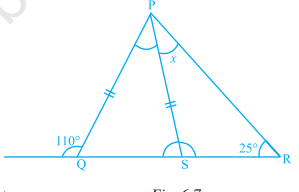Solution:-

(b) 45o

From the given figure,

In triangle PQS, ∠PSQ + ∠QPS = 110o … [from exterior angle property of a triangle]

We know that, sum of all angles of the triangle is equal to 180o.

So, ∠PSQ + ∠QPS + ∠PQS = 180o

∠PQS = 180o – 110o

∠PQS = 70o

Now, consider the triangle PRS,

∠PSQ = x + 25o … [from the exterior angle property of a triangle]

x = 70o – 25o

x = 45o

4. In a right-angled triangle, the angles other than the right angle are

(a) obtuse (b) right (c) acute (d) straight

Solution:-

(c) acute

5. In an isosceles triangle, one angle is 70°. The other two angles are of

(i) 55° and 55° (ii) 70° and 40° (iii) any measure

In the given option(s) which of the above statement(s) are true?

(a) (i) only (b) (ii) only (c) (iii) only (d) (i) and(ii)

Solution:-

(d) (i) and(ii)

From the question it is given that,

One angle of an isosceles triangle is 70o.

We know that, in an isosceles triangle 2 angles are equal corresponding with 2 equal sides,

If 70o is 3rd angle of triangle,

70o + x + x = 180o

2x + 70o = 180o

2x = 180o – 70o

x = (110o/2)

x = 55o

So, both angles are 55o

Consider the 70o as base angle of an isosceles triangle

Then, 70o + 70o + x = 180o

x = 180o – 140o

x = 40o

So, one angle is 40o and another is 70o

6. In a triangle, one angle is of 90°. Then

(i) The other two angles are of 45° each

(ii) In remaining two angles, one angle is 90° and other is 45°

(iii) Remaining two angles are complementary In the given option(s) which is true?

(a) (i) only (b) (ii) only (c) (iii) only (d) (i) and (ii)

Solution:-

(c) (iii) only

7. Lengths of sides of a triangle are 3 cm, 4 cm and 5 cm. The triangle is

(a) Obtuse angled triangle (b) Acute-angled triangle

(c) Right-angled triangle (d) An Isosceles right triangle

Solution:-

(c) Right-angled triangle

8. In Fig. 6.8, PB = PD. The value of x is

(a) 85o (b) 90o (c) 25o (d) 35o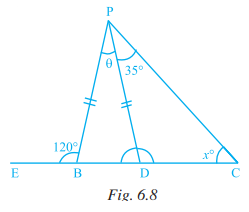Solution:-

(c) 25o

Exterior angle of triangle is equal to sum of 2 opposite interior angles.

As BC is straight line

∠PBD + 120° = 180°

∠PBD = 180° – 120°

∠PBD = 60°

Given in an isosceles ΔPBD, PB = PD

∴ ∠PBD = ∠PDB

With exterior angle ∠PSQ equal to sum of opposite interior angles

∠PDB = ∠DPC + ∠PCD [Exterior Angle Property]

60° = x + 35°

x = 60° – 35°

x = 25°

9. In ∆PQR,

(a) PQ – QR > PR

(b) PQ + QR < PR

(c) PQ – QR< PR

(d) PQ + PR< QR

Solution:-

(c) PQ – QR < PR

The difference of the lengths of any two sides of a triangle is always smaller than the length of the third side.

10. In ∆ ABC,

(a) AB + BC > AC

(b) AB + BC < AC

(c) AB + AC < BC

(d) AC + BC < AB

Solution:-

(a) AB + BC > AC

The sum of the lengths of any two sides of a triangle is always greater than the length of the third side.

11. The top of a broken tree touches the ground at a distance of 12 m from its base. If the tree is broken at a height of 5 m from the ground then the actual height of the tree is

(a) 25 m (b) 13 m (c) 18 m (d) 17 m

Solution: –

(c) 18 m

From the question it is given that,

The top of a broken tree touches the ground at a distance of 12 m from its base

The broken height of the tree = 5 m

By using Pythagoras theorem,

Hypotenuse2 = Base2 + Height2

Hypotenuse2 = 122 + 52

Hypotenuse2 = 144 + 25

Hypotenuse2 = 169

Hypotenuse = √169

Hypotenuse = 13

So, the total height of tree = 5 + 13 = 18 m

12. The triangle ABC formed by AB = 5 cm, BC = 8 cm, AC = 4 cm is

(a) an isosceles triangle only (b) a scalene triangle only

(c) an isosceles right triangle (d) scalene as well as a right triangle

Solution: –

(b) a scalene triangle only

A scalene triangle is a triangle that has three unequal sides.

13. Two trees 7 m and 4 m high stand upright on a ground. If their bases (roots) are 4 m apart, then the distance between their tops is

(a) 3 m (b) 5 m (c) 4 m (d) 11 m

Solution:-

(b) 5 m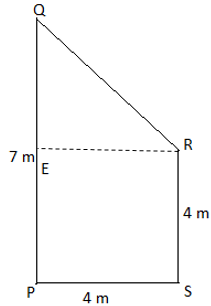Consider PQ is the tree of height 7m and RS is the tree of height 4 m.

So, consider the triangle QRE, from the Pythagoras theorem,

QR2 = QE2 + ER2

QR2 = 32 + 42

QR2 = 9 + 16

QR2 = 25

QR = √25

QR = 5

Therefore, the distance between the top of the two trees is 5m.

14. If in an isosceles triangle, each of the base angles is 40°, then the triangle is

(a) Right-angled triangle (b) Acute angled triangle

(c) Obtuse angled triangle (d) Isosceles right-angled triangle

Solution: –

(c) Obtuse angled triangle

We know that, sum of interior angles of triangle is equal to 180o.

Let us assume the 3rd angle be Q,

Then, 40o + 40o + Q = 180o

80o + Q = 180o

Q = 180 – 80

Q = 100o

An obtuse triangle (or obtuse-angled triangle) is a triangle with one obtuse angle (greater than 90°) and two acute angles. Since a triangle’s angles must sum to 180o.

15. If two angles of a triangle are 60° each, then the triangle is

(a) Isosceles but not equilateral (b) Scalene

(c) Equilateral (d) Right-angled

Solution:-

(c) Equilateral

In an equilateral triangle, each angle has measure 60o.

16. The perimeter of the rectangle whose length is 60 cm and a diagonal is 61 cm is

(a) 120 cm (b) 122 cm (c) 71 cm (d) 142 cm

Solution:-

(d) 142 cm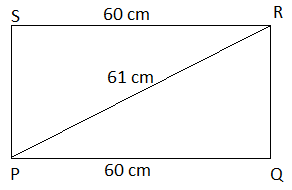Consider the rectangle PQRS,

Given, length of rectangle PQ = 60 cm, Diagonal of the rectangle = 61 cm.

To find out the height of the rectangle, consider the right angled triangle PQR.

From the Pythagoras theorem, PR2 = PQ2 + RQ2

612 = 602 + RQ2

3721 = 3600 + RQ2

RQ2 = 3721 – 3600

RQ2 = 121

RQ = √121

RQ = 11 cm

Then, the perimeter of the rectangle PQRS = 2 (Length + Breadth)

= 2 (60 + 11)

= 2 (71)

= 142 cm

17. In ∆PQR, if PQ = QR and ∠Q = 100o, then ∠R is equal to

(a) 40o (b) 80o (c) 120o (d) 50o

Solution: –

(a) 40o

Given, In ∆PQR, PQ = QR so it is an isosceles triangle.

Then, ∠P = ∠R

So, let us assume two angles be x

x + x + 100o = 180o

2x = 180o – 100o

2x = 80o

x = 80o/2

x = 40o

Therefore, x = ∠P = ∠R = 40o

18. Which of the following statements is not correct?

(a) The sum of any two sides of a triangle is greater than the third side

(b) A triangle can have all its angles acute

(c) A right-angled triangle cannot be equilateral

(d) Difference of any two sides of a triangle is greater than the third side

Solution: –

(d) Difference of any two sides of a triangle is greater than the third side.

The difference of the lengths of any two sides of a triangle is always smaller than the length of the third side.

19. In Fig. 6.9, BC = CA and ∠A = 40. Then, ∠ACD is equal to

(a) 40o (b) 80o (c) 120o (d) 60o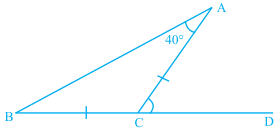Solution:-

(b) 80o

We know that, the exterior angle is equal to sum of opposite interior angles.

So, ∠ACD = ∠A + ∠B

As ΔACB is an isosceles triangle with AC = BC

Therefore, ∠A must be equal to ∠B

∠ACD = 40o + 40o

= 80o

20. The length of two sides of a triangle are 7 cm and 9 cm. The length of the third side may lie between

(a) 1 cm and 10 cm

(b) 2 cm and 8 cm

(c) 3 cm and 16 cm

(d) 1 cm and 16 cm

Solution: –

(c) 3 cm and 16 cm

From the question it is given that, the length of two sides of a triangle are 7 cm and 9 cm.

Let us assume the length of the third side of the triangle be ‘P’.

We Know that, the sum of the two sides of the triangle is greater than the third side.

So, 7 + 9 > P

16 > P

Now, difference between two sides = 9 – 7 = 2

Therefore, the third side is greater than 2 and smaller than 16.

i.e. 3 cm and 16 cm

21. From Fig. 6.10, the value of x is

(a) 75o (b) 90o (c) 120o (d) 60o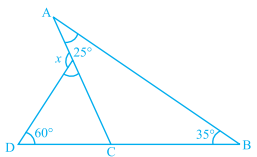Solution:-

(c) 120o

We know that, exterior angle is equal to sum of opposite interior angles.

From the figure,

∠ACD = ∠A + ∠B

∠ACD = 25o + 35o

= 60°

Then, in another triangle

x is exterior angle

∴ x = 60° + ∠ACD

x = 60° + 60°

x = 120°

22. In Fig. 6.11, the value of ∠A + ∠B + ∠C + ∠D + ∠E + ∠F is

(a) 190o (b) 540o (c) 360o (d) 180o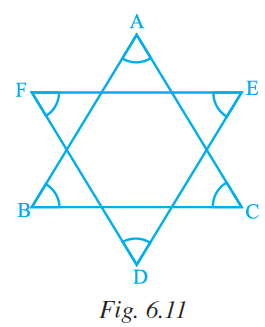Solution: –

(c) 360o

From the figure, we can able to find out there are two triangles.

So, consider the ΔABC,

We know that, sum of the interior angles of the triangle is equal to 180o.

Therefore, ∠A + ∠B + ∠C = 180o

Now, consider the ΔDEF,

∠D + ∠E + ∠F = 180o

Then,

= ∠A + ∠B + ∠C + ∠D + ∠E + ∠F

= 180o + 180o

= 360o

23. In Fig. 6.12, PQ = PR, RS = RQ and ST || QR. If the exterior angle RPU is 140°, then the measure of angle TSR is

(a) 55o (b) 40o (c) 50o (d) 45o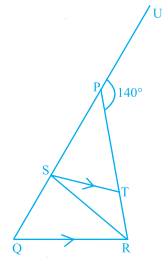Solution:-

(b) 40o

Consider the ΔPQR.

From the exterior angle property

∠RPU = ∠PRQ + ∠PQR

140O = 2 ∠PQR … [given PQ = PR]

∠PQR = 140/2

∠PQR = 70o

Given, ST || QR and QS is transversal.

From the property of corresponding angles, ∠PST = ∠PQR = 70o

Now, consider the ΔQSR

RS = RQ … [from the question]

So, ∠SQR = ∠RSQ = 70O

Then, PQ is a straight line.

∠PST + ∠TSR + ∠RSQ = 180o

70o + ∠TSR + 70o = 180o

140o + ∠TSR = 180o

∠TSR = 180o – 140o

∠TSR = 40o

24. In Fig. 6.13, ∠BAC = 90°, AD ⊥ BC and ∠BAD = 50°, then ∠ACD is

(a) 50° (b) 40° (c) 70° (d) 60°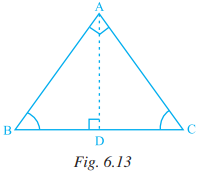Solution:-

(a) 50o

From the question it is given that, ∠BAC = 90o AD ⊥ BC and ∠BAD = 50o

So, ∠DAC = ∠BAC – ∠BAD

= 90o – 50o

= 40o

From the rule of exterior angle property = ∠ADB = ∠DAC + ∠ACD

90o = 40o + ∠ACD

∠ACD = 90 – 40

∠ACD = 50o

25. If one angle of a triangle is equal to the sum of the other two angles, the triangle is (a) obtuse (b) acute (c) right (d) equilateral

Solution:-

(c) right

26. If the exterior angle of a triangle is 130° and its interior opposite angles are equal, then measure of each interior opposite angle is

(a) 55o (b) 65o (c) 50o (d) 60o

Solution:-

(b) 65o

Let us assume the interior opposite angles are Q and Q.

Then, 130o = Q + Q … [from exterior angle property]

2Q = 130o

Q = 130o/2

Q = 65o

Therefore, the measure of each interior opposite angle is 65o.

27. If one of the angles of a triangle is 110°, then the angle between the bisectors of the other two angles is

(a) 70o (b) 110o (c) 35o (d) 145o

Solution: –

(d) 145o

From the question it is given that, one of the angles of triangle is 110o

We know that, sum of all angles of triangle is equal to 180o.

So, sum of other 2 angles is 180o – 110o = 70o

Then, both angles get halved 70o/2 = 35o

Sum of bisected angles will be half of sum of angles of triangle.

Then, the third angle will be = 180o – 35o

= 145o

28. In ∆ABC, AD is the bisector of ∠A meeting BC at D, CF ⊥ AB and E is the mid-point of AC. Then median of the triangle is

(a) AD (b) BE (c) FC (d) DE

Solution: –

(b) BE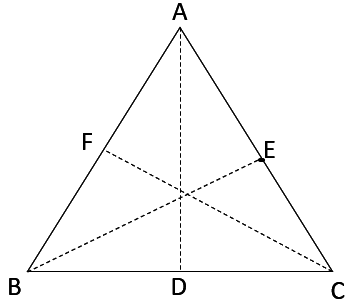We know that the median of triangle divides the opposite side into two equal parts.

Hence, BE is the median (as AE = EC).

29. In ∆PQR, if ∠ P = 60o, and ∠ Q = 40o, then the exterior angle formed by producing QR is equal to

(a) 60o (b) 120o (c) 100o (d) 80o

Solution:-

(c) 100o

As we know that, exterior angle is sum of opposite interior angles.

Then, the exterior angle formed by producing QR

It has opposite interior angles ∠P and ∠Q

Therefore, exterior angle = ∠P + ∠Q

= 60o + 40o

= 100o

30. Which of the following triplets cannot be the angles of a triangle?

(a) 67°, 51°, 62° (b) 70°, 83°, 27°

(c) 90°, 70°, 20° (d) 40°, 132°, 18°

Solution: –

(d) 40°, 132°, 18°

We know that, sum of angles of triangle is equal to 180o.

But, 40o + 132o + 18o = 190

So, these triplets cannot be the angles of a triangle.

31. Which of the following can be the length of the third side of a triangle whose two sides measure 18 cm and 14 cm?

(a) 4 cm (b) 3 cm (c) 5 cm (d) 32 cm

Solution:-

(c) 5 cm

We know that,

The sum of the lengths of any two sides of a triangle is always greater than the length of the third side.

So, 18 cm + 14 cm > 3rd side

3rd side < 32 cm

The difference of the lengths of any two sides of a triangle is always smaller than the length of the third side.

So, 18 – 14 < 3rd side

3rd side > 4 cm

Therefore, 5 cm is the length of the 3rd side.

32. How many altitudes does a triangle have?

(a) 1 (b) 3 (c) 6 (d) 9

Solution: –

(b) 3

The perpendicular line segment from a vertex of a triangle to its opposite side is called an altitude of the triangle. A triangle has 3 altitudes.

33. If we join a vertex to a point on opposite side which divides that side in the ratio 1:1, then what is the special name of that line segment?

(a) Median (b) Angle bisector (c) Altitude (d) Hypotenuse

Solution:-

(a) Median

The line segment joining a vertex of a triangle to the mid point of its opposite side is called a median of the triangle.

34. The measures of ∠x and ∠y in Fig. 6.14 are respectively

(a) 30°, 60° (b) 40°, 40°

(c) 70°, 70° (d) 70°, 60°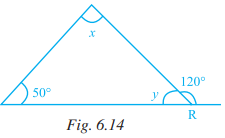Solution:-

(d) 70o, 60o

We know that, the exterior angle is sum of interior opposite angles of triangle.

So, x + 50o = 120o

x = 120o – 50o

x = 70o

We also know that, sum of angles of triangle are equal to 180o.

So, 50o + x + y = 180o

50o + 70o + y = 180o

120o + y = 180o

y = 180o – 120o

y = 60o

Therefore, the measures of ∠x and ∠y is 70o and 60o respectively.

35. If length of two sides of a triangle are 6 cm and 10 cm, then the length of the third side can be

(a) 3 cm (b) 4 cm (c) 2 cm (d) 6 cm

Solution: –

(d) 6 cm

We know that,

The sum of the lengths of any two sides of a triangle is always greater than the length of the third side.

So, 6 cm + 10 cm > 3rd side

3rd side < 16 cm

The difference of the lengths of any two sides of a triangle is always smaller than the length of the third side.

So, 10 – 6 < 3rd side

3rd side > 4 cm

Therefore, 6 cm is the length of the 3rd side.

36. In a right-angled triangle ABC, if angle B = 90°, BC = 3 cm and AC = 5 cm, then the length of side AB is

(a) 3 cm (b) 4 cm (c) 5 cm (d) 6 cm

Solution: –

(b) 4 cm

From Pythagoras theorem.

AC2 = AB2 + BC2

52 = AB2 + 32

AB2 = 25 – 9

AB2 = 16

AB = √16

AB = 4 cm

37. In a right-angled triangle ABC, if angle B = 90°, then which of the following is true? (a) AB2 = BC2 + AC2

(b) AC2 = AB2 + BC2

(c) AB = BC + AC

(d) AC = AB + BC

Solution:-

(b) AC2 = AB2 + BC2 … [from Pythagoras theorem]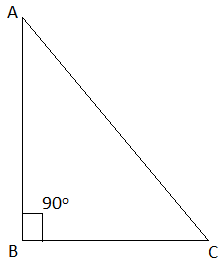38. Which of the following figures will have its altitude outside the triangle?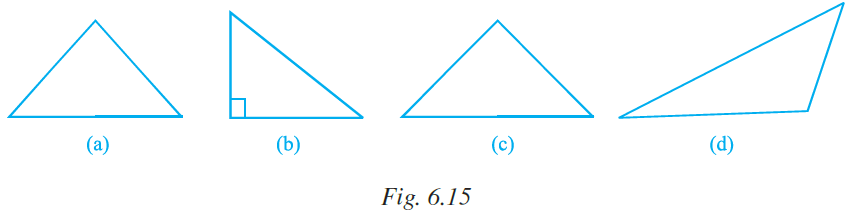Solution:-

Figure (d) has its altitude outside the triangle

39. In Fig. 6.16, if AB || CD, then,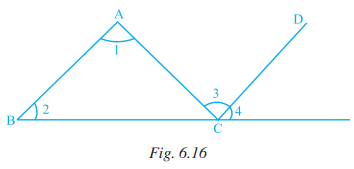(a) ∠ 2 = ∠ 3 (b) ∠ 1 = ∠ 4

(c) ∠ 4 = ∠ 1 + ∠ 2 (d) ∠ 1 + ∠ 2 = ∠ 3 + ∠ 4

Solution:-

(d) ∠ 1 + ∠ 2 = ∠ 3 + ∠ 4

As we know that, exterior angle is equal to the sum of opposite interior angles

Consider, Δ ABC

As BC is extended

∠A + ∠B = ∠3 + ∠4

Therefore, ∠1 + ∠2 = ∠3 + ∠4

40. In ∆ABC, ∠Α = 100°, AD bisects ∠A and AD⊥BC. Then, ∠B is equal to

(a) 80o (b) 20o (c) 40o (d) 30o

Solution: –

(c) 40o

Consider the triangle ABC,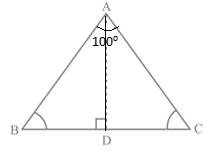From the figure, AD bisects ∠Α

∠DAC = 50o

∠ΑDC = 90o

Consider the ∆ABD,

From the rule of exterior angle property of triangle,

90o = ∠ΑBD + 50o

∠ΑBD = 90o – 50o

= 40o

41. In ∆ABC, ∠Α = 50°, ∠B = 70° and bisector of ∠C meets AB in D (Fig. 6.17). Measure of ∠ADC is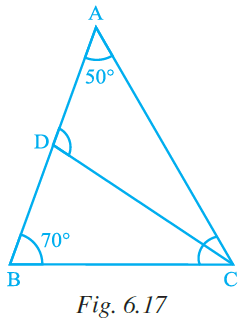(a) 50° (b) 100° (c) 30° (d) 70°

Solution:-

(b) 100o

From the figure,

Consider the ΔABC,

We know that, sum of angles of triangle is equal to 180o.

So, ∠Α + ∠B + ∠C = 180o

50o + 70o + ∠C = 180o

∠C + 120o = 180o

∠C = 180o – 120o

∠C = 60o

Since CD bisects ∠C,

So, ∠DCB = ∠ΑCD = ½ ∠C

= 60o/2

= 30o

Now, consider ΔBDC

From exterior angle property, ∠ADC = ∠DBC + ∠DCB

= 100o

42. If for ∆ABC and ∆DEF, the correspondence CAB ↔ EDF gives a congruence, then which of the following is not true?

(a) AC = DE (b) AB = EF (c) ∠A = ∠D (d) ∠C = ∠E

Solution: –

(b) AB = EF

Because, for ∆ABC and ∆DEF AB = DF

43. In Fig. 6.18, M is the mid-point of both AC and BD. Then

(a) ∠1 = ∠2 (b) ∠1 = ∠4 (c) ∠2 = ∠4 (d) ∠1 = ∠3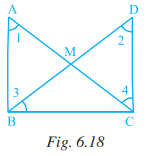Solution: –

(b) ∠1 = ∠4

From the figure, M is the mid-point of both AC and BD.

By the corresponding parts of congruent triangles, ∠1 = ∠4.

44. If D is the mid-point of the side BC in ∆ABC where AB = AC, then ∠ADC is

(a) 60° (b) 45° (c) 120s° (d) 90°

Solution:-

(d) 90o

We know that, in an isosceles triangle altitude and median are the same.

From the question, if D is the mid-point of the side BC in ∆ABC

Where, D is midpoint of BC joining from point A gives AD as median.

It possess 90o angle on BC

45. Two triangles are congruent, if two angles and the side included between them in one of the triangles are equal to the two angles and the side included between them of the other triangle. This is known as the

(a) RHS congruence criterion

(b) ASA congruence criterion

(c) SAS congruence criterion

(d) AAA congruence criterion

Solution:-

(b) ASA congruence criterion

46. By which congruency criterion, the two triangles in Fig. 6.19 are congruent?

(a) RHS (b) ASA (c) SSS (d) SAS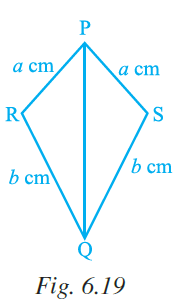Solution:-

(c) SSS

Under a given correspondence, two triangles are congruent, if the three sides of the one are equal to the three sides of the other.

47. By which of the following criterion two triangles cannot be proved congruent?

(a) AAA (b) SSS (c) SAS (d) ASA

Solution: –

(a) AAA

In AAA criterion two triangles cannot be proved congruent.

48. If ∆PQR is congruent to ∆STU (Fig. 6.20), then what is the length of TU?

(a) 5 cm (b) 6 cm (c) 7 cm (d) cannot be determined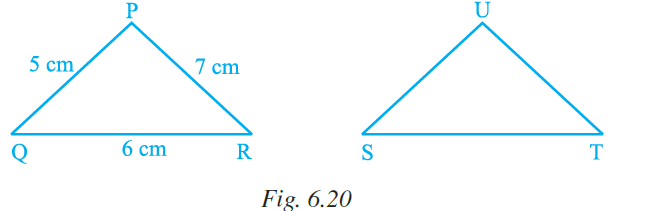Solution:-

(b) 6 cm

From the question it is given that,

Δ PQR ≅ Δ STU

So,

PQR ↔ STU

∴ QR = TU

TU = 6cm

49. If ∆ABC and ∆DBC are on the same base BC, AB = DC and AC = DB (Fig. 6.21), then which of the following gives a congruence relationship?

(a) ∆ ABC ≅ ∆ DBC (b) ∆ ABC ≅ ∆CBD

(c) ∆ ABC ≅ ∆ DCB (d) ∆ ABC ≅ ∆BCD

Solution:-

(c) ∆ ABC ≅ ∆ DCB

Consider the ΔABC and ΔDCB,

From the question it is given that, AB = DC and AC = DB

BC = BC … [because common side]

Therefore, ∆ ABC ≅ ∆ DCB

In questions 50 to 69, fill in the blanks to make the statements true.

50. The triangle always has altitude outside itself.

Solution:-

The Obtuse triangle always has altitude outside itself.

51. The sum of an exterior angle of a triangle and its adjacent angle is always .

Solution:-

The sum of an exterior angle of a triangle and its adjacent angle is always 1800.

52. The longest side of a right angled triangle is called its .

Solution: –

The longest side of a right angled triangle is called its hypotenuse.

53. Median is also called in an equilateral triangle.

Solution:-

Median is also called altitude in an equilateral triangle

54. Measures of each of the angles of an equilateral triangle is .

Solution: –

Measures of each of the angles of an equilateral triangle is 60o.

55. In an isosceles triangle, two angles are always .

Solution: –

In an isosceles triangle, two angles are always equal.

56. In an isosceles triangle, angles opposite to equal sides are .

Solution: –

In an isosceles triangle, angles opposite to equal sides are equal.

57. If one angle of a triangle is equal to the sum of other two, then the measure of that angle is .

Solution: –

If one angle of a triangle is equal to the sum of other two, then the measure of that angle is 90o.

58. Every triangle has at least acute angle (s).

Solution:

Every triangle has at least two acute angle (s).

59. Two line segments are congruent, if they are of lengths.

Solution: –

Two line segments are congruent, if they are of equal lengths.

60. Two angles are said to be , if they have equal measures.

Solution: –

Two angles are said to be congruent, if they have equal measures.

61. Two rectangles are congruent, if they have same and .

Solution: –

Two rectangles are congruent, if they have same and length and breadth.

62. Two squares are congruent, if they have same .

Solution:-

Two squares are congruent, if they have same side.

63. If ∆PQR and ∆XYZ are congruent under the correspondence QPR ↔ XYZ, then

(i) ∠R = (ii) QR = (iii) ∠P =

(iv) QP = (v) ∠ Q = (vi) RP =

Solution: –

If ∆PQR and ∆XYZ are congruent under the correspondence QPR ↔ XYZ, then

(i) ∠R = ∠Z (ii) QR = XZ (iii) ∠P = ∠Y

(iv) QP = XY (v) ∠Q = ∠X (vi) RP = ZY

64. In Fig. 6.22, ∆PQR ≅ ∆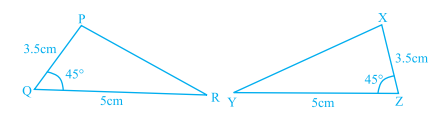Fig. 6.22

Solution: –

In Fig. 6.22, ∆PQR ≅ ∆XZY

From the figure, PQ = XZ = 3.5 cm

QR = ZY = 5cm

∠PQR = ∠XZY = 45o

From SAS criterion, ∆PQR ≅ ∆XZY

65. In Fig. 6.23, ∆PQR ≅ ∆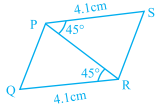Fig. 6.23

Solution: –

In Fig. 6.23, ∆PQR ≅ ∆RSP

From the figure, PS = RQ = 4.1 cm

PR = PR … [common side for both triangles]

∠PRQ = ∠RPS = 45o

From SAS criterion, ∆PQR ≅ ∆RSP

66. In Fig. 6.24, ∆ ≅ ∆ PQR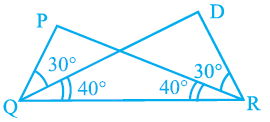Fig. 6.24

Solution:-

In Fig. 6.24, ∆DRQ ≅ ∆ PQR

From the figure,

QR = QR … [common side for both triangles]

∠PRQ = ∠DQR = 40o

∠PQR = ∠DRQ = 70o

From ASA criterion, ∆DRQ ≅ ∆ PQR

67. In Fig. 6.25, ∆ ARO ≅ ∆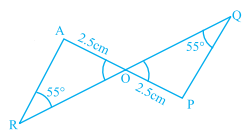Fig. 6.25

Solution:-

In Fig. 6.25, ∆ ARO ≅ ∆PQO

From the figure,

AO = PO = 2.5 cm

∠ARO = ∠PQO = 55o

∠AOR = ∠POQ [vertically opposite angles]

From ASA criterion, ∆ARO ≅ ∆ PQO

68. In Fig. 6.26, AB = AD and ∠BAC = ∠ DAC.

Then (i) ∆ ≅ ∆ ABC.

(ii) BC = .

(iii) ∠BCA = .

(iv) Line segment AC bisects and .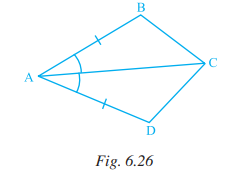Solution: –

In Fig. 6.26, AB = AD and ∠BAC = ∠ DAC.

Then (i) ∆ADC ≅ ∆ ABC.

(ii) BC = DC.

(iii) ∠BCA = ∠DCA.

(iv) Line segment AC bisects ∠BAD and ∠BCD.

69. In Fig. 6.27,

(i) ∠ TPQ = ∠ _____ + ∠ _____

(ii) ∠ UQR = ∠ _____ + ∠ _____

(iii) ∠ PRS = ∠ _____ + ∠ _____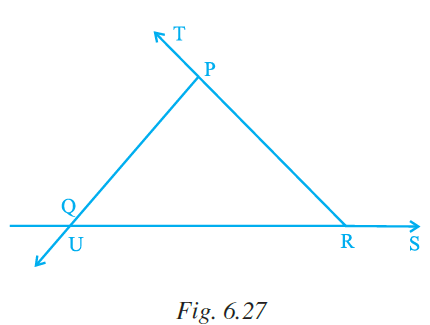Fig. 6.27

Solution: –

In Fig. 6.27,

(i) ∠ TPQ = ∠ PQR + ∠ PRQ … [from exterior angle property]

(ii) ∠ UQR = ∠ QRP + ∠ QPR … [from exterior angle property]

(iii) ∠ PRS = ∠ RPQ + ∠ RQP … [from exterior angle property]

In questions 70 to 80 state whether the statements are True or False.

70. In a triangle, sum of squares of two sides is equal to the square of the third side.

Solution: –

False

In a right angled triangle, sum of squares of two sides is equal to the square of the third side.

71. Sum of two sides of a triangle is greater than or equal to the third side.

Solution: –

False

The sum of the lengths of any two sides of a triangle is always greater than the length of the third side.

72. The difference between the lengths of any two sides of a triangle is smaller than the length of third side.

Solution: –

True.

73. In ∆ABC, AB = 3.5 cm, AC = 5 cm, BC = 6 cm and in ∆PQR, PR= 3.5 cm, PQ = 5 cm, RQ = 6 cm. Then ∆ABC ≅ ∆PQR.

Solution: –

False

In ∆ABC, AB = 3.5 cm, AC = 5 cm, BC = 6 cm and in ∆PQR, PR= 3.5 cm, PQ = 5 cm, RQ = 6 cm. Then ∆ABC ≅ ∆PRQ

74. Sum of any two angles of a triangle is always greater than the third angle.

Solution: –

False

Sum of any two angles of a triangle is either greater than the third angle or smaller than the third angle.

75. The sum of the measures of three angles of a triangle is greater than 180o.

Solution: –

False

The sum of the measures of three angles of a triangle is equal to 180o.

76. It is possible to have a right-angled equilateral triangle.

Solution: –

False

In a right angled triangle, sum of squares of two sides is equal to the square of the third side.

But, in equilateral triangle all sides are always equal.

77. If M is the mid-point of a line segment AB, then we can say that AM and MB are congruent.

Solution: –

True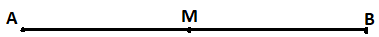In the figure, M is the midpoint,

So, AM = MB

78. It is possible to have a triangle in which two of the angles are right angles.

Solution: –

False.

It is not possible to have a triangle in which two of the angles are right angles.

79. It is possible to have a triangle in which two of the angles are obtuse.

Solution: –

False.

It is not possible to have a triangle in which two of the angles are obtuse

80. It is possible to have a triangle in which two angles are acute.

Solution: –

True.